# Wolfram Physics Project Bulletins

PRELIMINARY VERSION

# A Short Note on the Double-Slit Experiment and Other Quantum Interference Effects in the Wolfram Model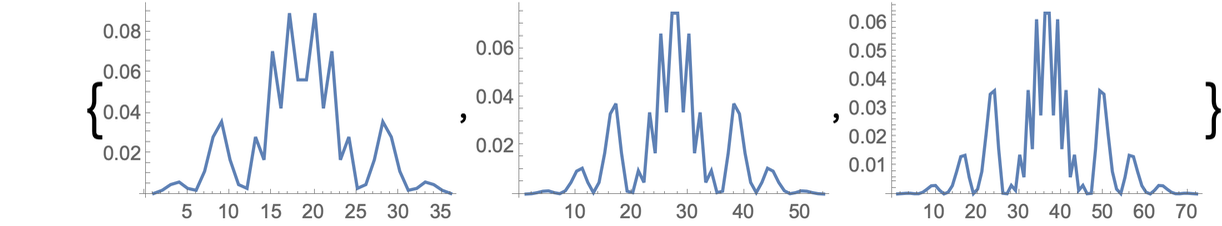This bulletin is a short note detailing how single-slit, double-slit and multi-slit photon diffraction and interference patterns can be successfully reproduced using the author’s own formulation of quantum mechanics in the Wolfram model. The author has benefited greatly from many fruitful conversations with Stephen Wolfram, as well as from the encouragement (and infectious enthusiasm) of Hatem Elshatlawy.

## Introduction

When we announced the Wolfram Physics Project back in April, among the many launch documents that Stephen and I wrote was a paper in which I outlined a mathematical formulation of quantum mechanics in the Wolfram model in terms of multiway systems, path weights and completion procedures, and presented rigorous derivations of several key features of conventional quantum mechanical formalism, including the canonical commutation relations, the path integral and the Schrödinger equation. It even conjectured a precise algorithmic procedure by which one could describe the otherwise mysterious phenomenon of “wavefunction collapse” in the context of quantum measurement. It constituted a natural supplement to Stephen’s less mathematical (and correspondingly much more accessible) technical introduction to our quantum mechanical formalism. I outlined in great detail how quantum amplitudes would emerge as a consequence of path weights in the multiway evolution graph, with phase differences between pairs of paths thus corresponding to the ratios of branchlike- to spacelike-separated events, in such a way that applying an appropriate Knuth–Bendix completion procedure to the multiway system would force the analog of both constructive and destructive interference between different branches of history, exactly as conventional quantum mechanics predicts.

Since many of the key “classic” phenomena of quantum mechanics, such as the diffraction and interference of photons passing through parallel slits, are ultimately just elementary corollaries of this derivation of the Schrödinger equation, I fully expected that very soon after the release of my paper, somebody out there would take the requisite couple of minutes to sit down and write the trivial piece of code needed to reproduce the famous double-slit experiment in the Wolfram model. Following Stephen’s earnest advice to me about how best to instigate a new research program (“You mustn’t pick all the low-hanging fruit yourself! Leave something for the new people to do!”), I had explicitly decided to leave this particularly juicy-looking piece of fruit unpicked, hoping that some young student would come along and be the first to see the interference fringes for themselves. Several months went by, and despite my continued encouragement to various people (including several students at our Summer School!), for whatever reason, nobody did the experiment. So, in the end, I decided that I would just do it myself over a spare weekend, and this short bulletin was the result.

The first part of this bulletin demonstrates how, using only a simple string multiway system equipped with elementary path weights, one can easily reproduce the known phenomena of single-slit, double-slit and multi-slit photon interference, yielding intensity patterns that can be shown to converge to the results predicted analytically by the standard equations of optics/quantum mechanics, and indicates how these interference patterns connect to the geometry of branchial space (and hence to the geometry of the associated projective Hilbert space of the system). The second part then illustrates precisely why these interference patterns appear, as a straightforward consequence of the basic combinatorics of multiway systems and some elementary number theory; it demonstrates how the setup of the string multiway systems shown in the first part effectively encodes a position basis that maps points in branchial space onto corresponding points in physical space, it gives a minimal explicit example of pairs of interacting quantum oscillators to show directly how both constructive and destructive interference effects work within the multiway Wolfram model formalism, and it demonstrates precisely why these interference effects occur, as a consequence of some basic modular arithmetic and the combinatorial consequences of multiway completion rules. In effect, this bulletin may be considered to be a concrete computational instantiation of one of the somewhat more abstract (and correspondingly much more general) mathematical arguments presented within my previous quantum mechanics paper.

## The Big Result: Diffraction and Interference Patterns from Simple Multiway Systems

In conventional optics, diffraction and interference patterns are governed (at least in the case of near-field Fresnel diffraction phenomena, which is the case that we consider in this bulletin) by the transcendental Fresnel integrals, which can in turn be approximated by products of Chebyshev polynomials (of the second kind) with the Sinc function. As such, we can reproduce the spatial intensity plots for the standard single-slit, double-slit and triple-slit interference fringes in the following straightforward way: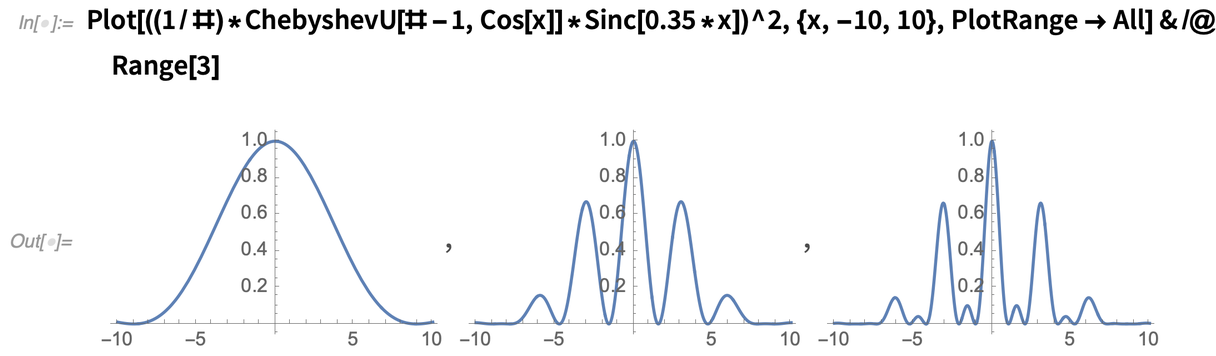✕ ```Plot[((1/#)*ChebyshevU[# - 1, Cos[x]]*Sinc[0.35*x])^2, {x, -10, 10}, PlotRange -> All] & /@ Range```

In my previous paper on the quantum mechanical foundations of the Wolfram model, I provided a rigorous mathematical formulation of the magnitudes of quantum amplitudes (in terms of path weights in multiway systems), and of quantum phases (in terms of ratios of branchlike- to spacelike-separated events in the multiway causal graph). Thus, an obvious question to ask would be whether we can successfully reproduce these conventional interference patterns using pure string-based multiway systems? Rather gratifyingly, it turns out that the answer is yes: diffraction and interference phenomena essentially “fall out” of my multiway formulation of quantum mechanics in an exceptionally natural way, as we shall now see.

Consider the following elementary string multiway system: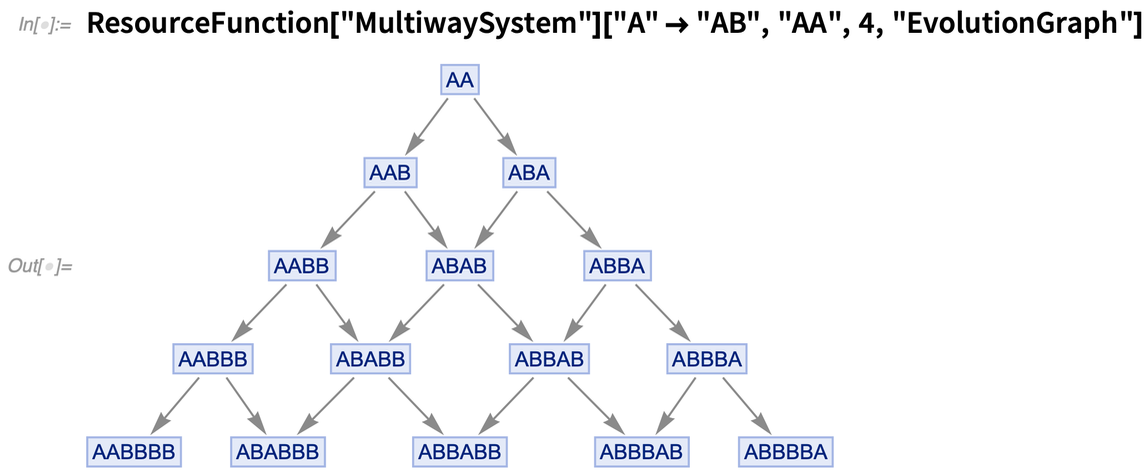✕ ```ResourceFunction["MultiwaySystem"][ "A" -> "AB", "AA", 4, "EvolutionGraph"]```

It is possible to introduce so-called “path weights” for each vertex in this system, such that every vertex is weighted by the number of distinct evolution paths that lead to it, as follows: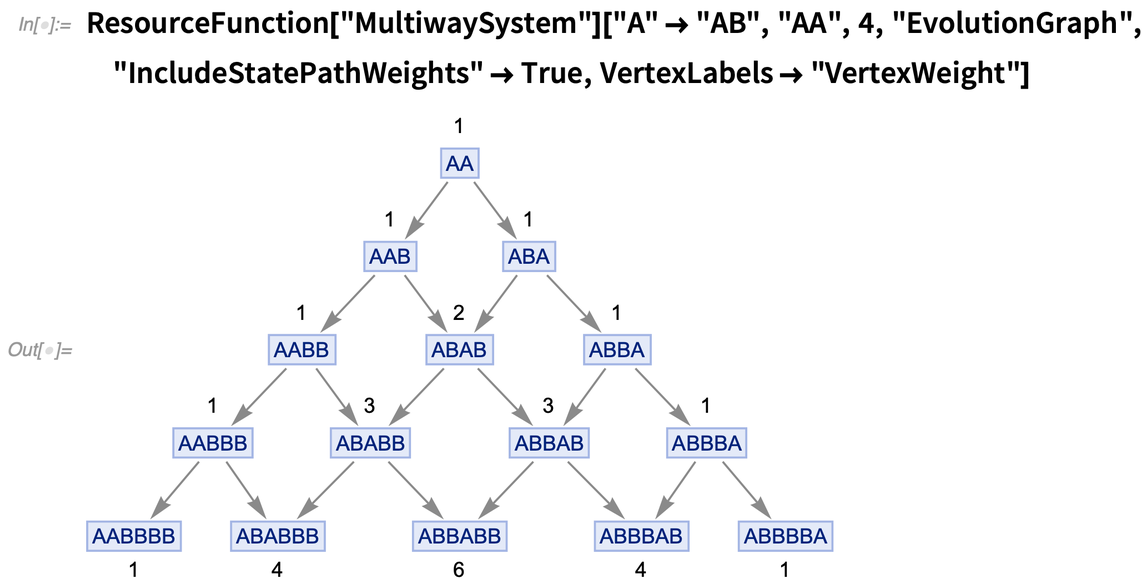✕ ```ResourceFunction["MultiwaySystem"][ "A" -> "AB", "AA", 4, "EvolutionGraph", "IncludeStatePathWeights" -> True, VertexLabels -> "VertexWeight"]```

From here, we can now “normalize” these path weights by imposing the constraint that all path weights for state vertices produced at a given step in the multiway evolution must sum to 1, like so: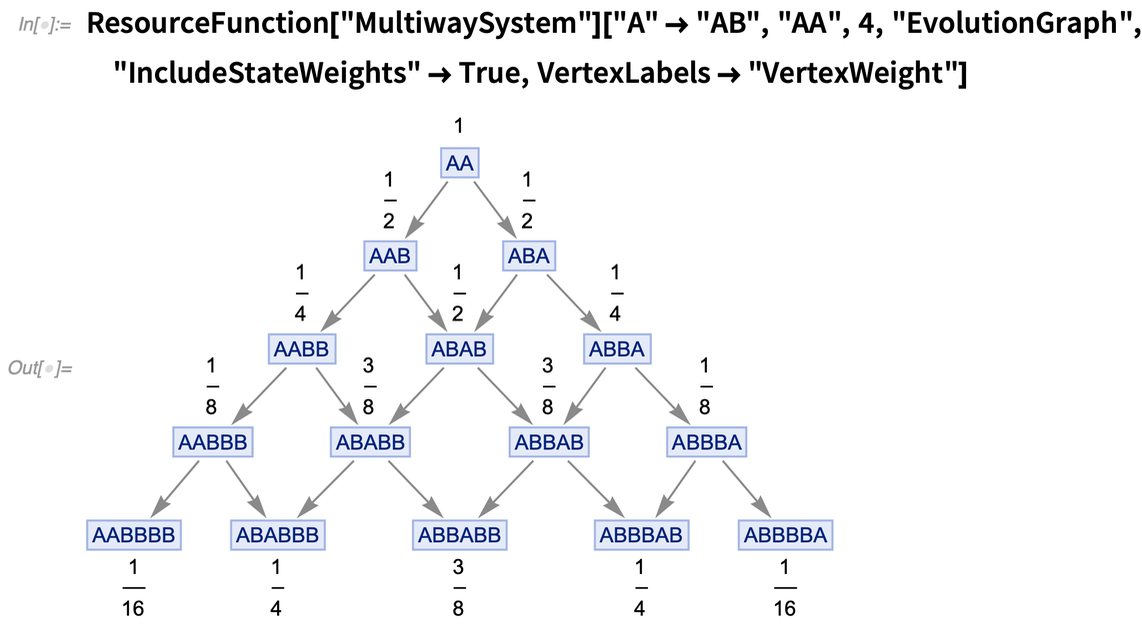✕ ```ResourceFunction["MultiwaySystem"][ "A" -> "AB", "AA", 4, "EvolutionGraph", "IncludeStateWeights" -> True, VertexLabels -> "VertexWeight"]```

And then, within my formulation of quantum mechanics, in which each global multiway state corresponds to a distinct eigenstate of the universe (i.e. as part of some generalized Hartle–Hawking wavefunction), these normalized state weights correspond neatly to the magnitudes (squared) of the quantum amplitudes for the associated eigenstates.

Now let us construct a toy example of a photon diffraction experiment using an elementary string multiway system; in a very loose sense, we could use the character “X” to denote the “presence” of a photon, and the character “o” to denote the “absence” of a photon, within a given region of space (although precisely how to construct a mathematically consistent position basis from these substrings is a somewhat subtle problem, as will be discussed later in detail in the second part of this bulletin). From here, we can represent a simple approximation to the Huygens–Fresnel principle of wave propagation using the sorting rules “Xo”  “oX” and “oX”  “Xo”, corresponding to a photon scattering right and scattering left, respectively. If we use the initial condition “ooooooooXoooooooo” to denote a single photon entering through a single slit, we therefore obtain the following multiway evolution: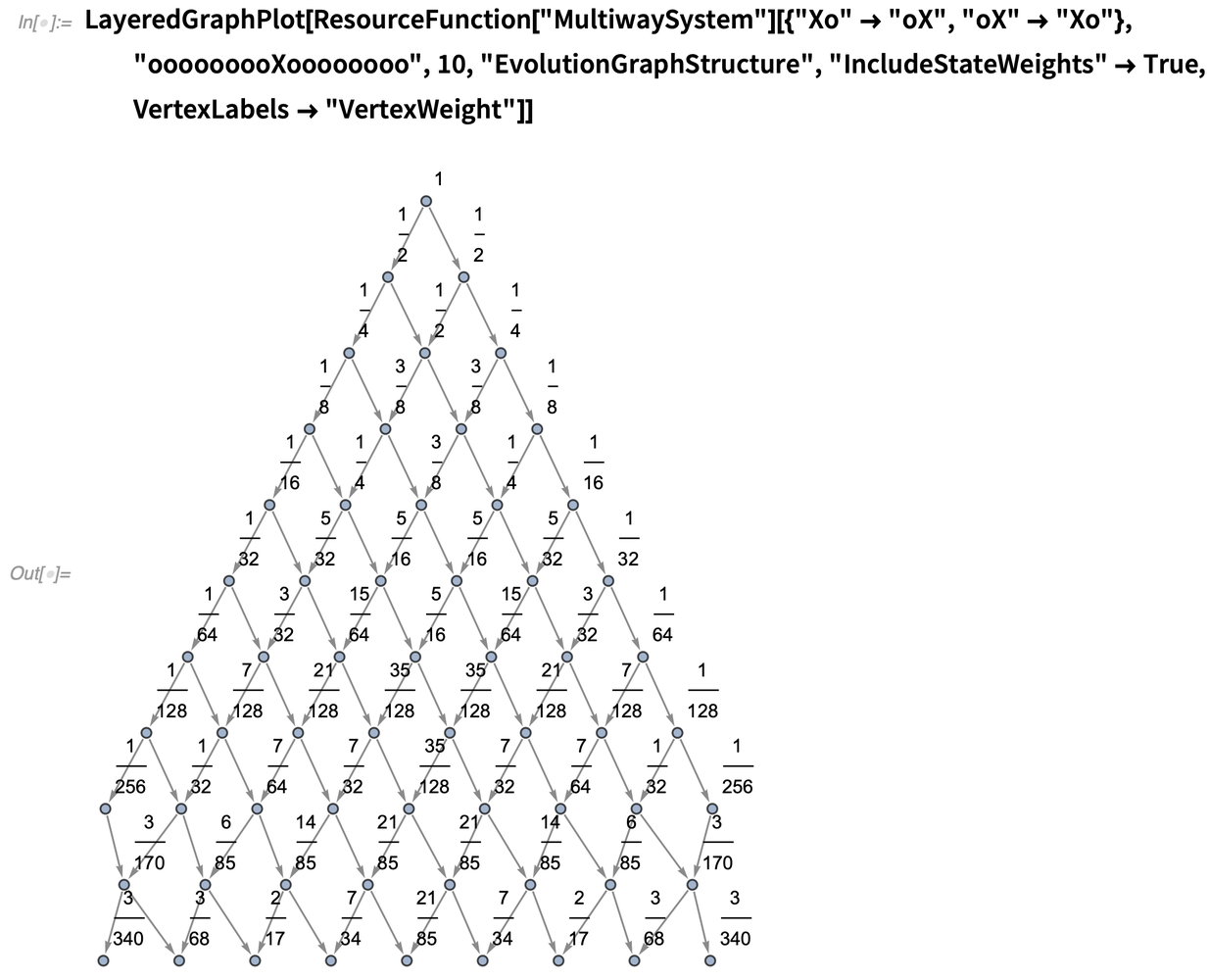✕ ```LayeredGraphPlot[ ResourceFunction["MultiwaySystem"][{"Xo" -> "oX", "oX" -> "Xo"}, "ooooooooXoooooooo", 10, "EvolutionGraphStructure", "IncludeStateWeights" -> True, VertexLabels -> "VertexWeight"]]```

We can see that there are exactly nine distinct states present at the final evolution step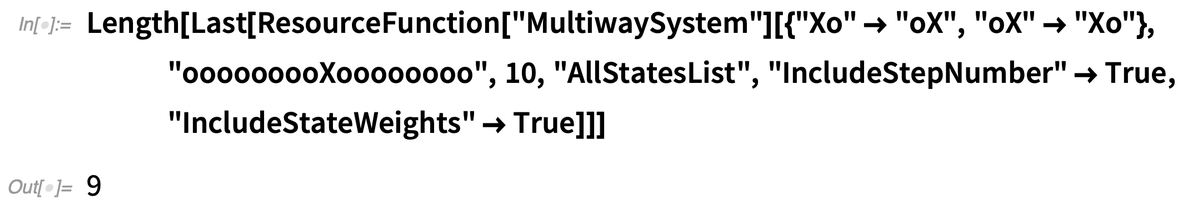✕ ```Length[Last[ ResourceFunction["MultiwaySystem"][{"Xo" -> "oX", "oX" -> "Xo"}, "ooooooooXoooooooo", 10, "AllStatesList", "IncludeStepNumber" -> True, "IncludeStateWeights" -> True]]]```

which means that we can extract the last nine vertex weights (which, due to the automatic string sorting performed by the MultiwaySystem function, have been naturally laid out from left to right in the appropriate “position basis”):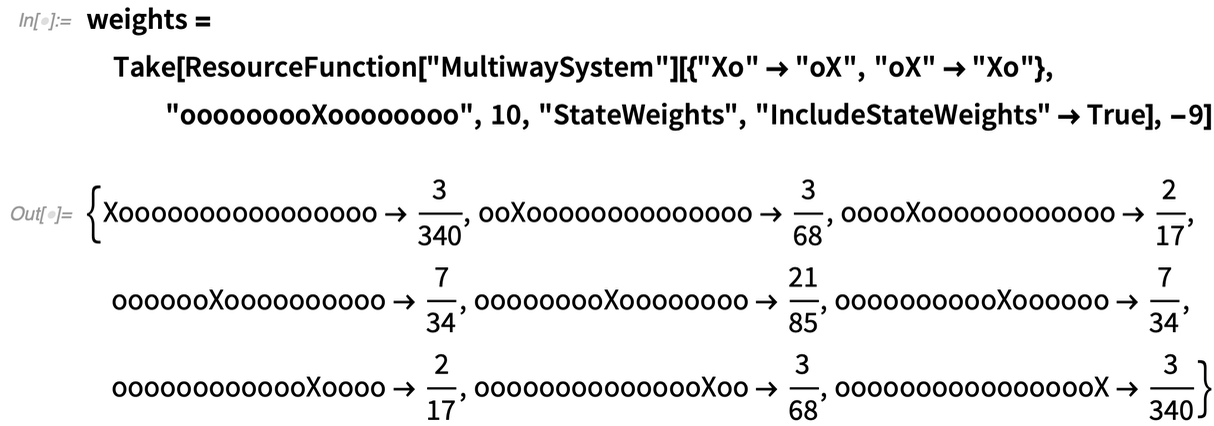✕ ```weights = Take[ResourceFunction["MultiwaySystem"][{"Xo" -> "oX", "oX" -> "Xo"}, "ooooooooXoooooooo", 10, "StateWeights", "IncludeStateWeights" -> True], -9]```

Thanks to the symmetry of the string sort, we can actually just plot the last half (5) of the vertex weights, so as to show the intensity pattern on the right-hand side of the fictional experimental “screen”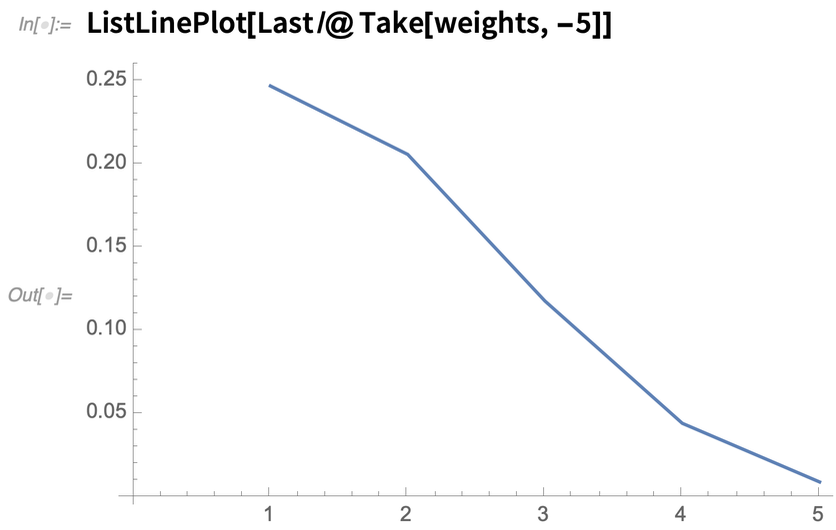✕ `ListLinePlot[Last /@ Take[weights, -5]]`

and then prepend a swapped version of the last half in order to reconstruct the pattern on the left-hand side: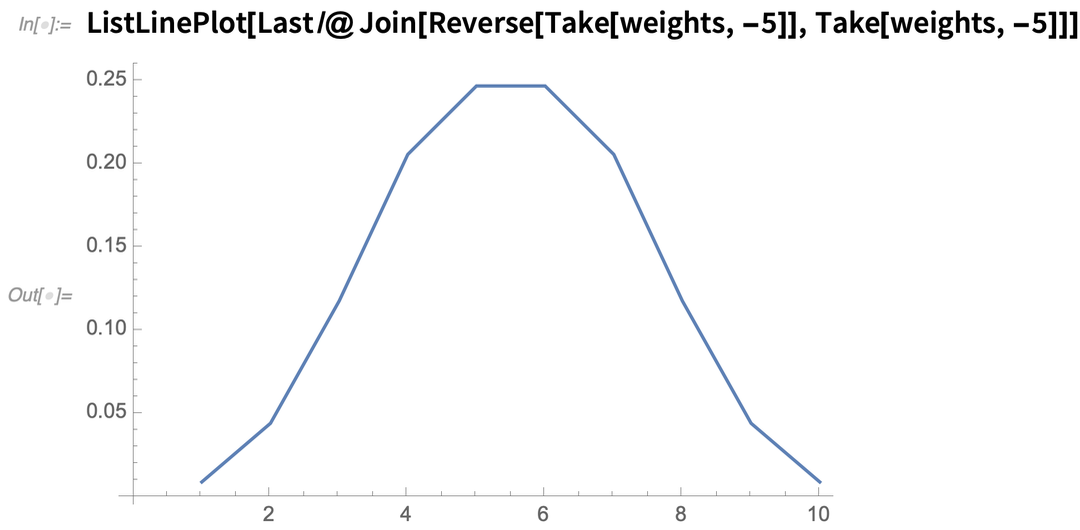✕ ```ListLinePlot[ Last /@ Join[Reverse[Take[weights, -5]], Take[weights, -5]]]```

So is this a single-slit diffraction pattern? It’s a little hard to tell with so few states, but it certainly seems suggestive of one. Let’s try the same procedure again with a larger initial condition (“ooooooooooooooooXoooooooooooooooo”) and more evolution steps, leading to the following (somewhat larger) multiway evolution graph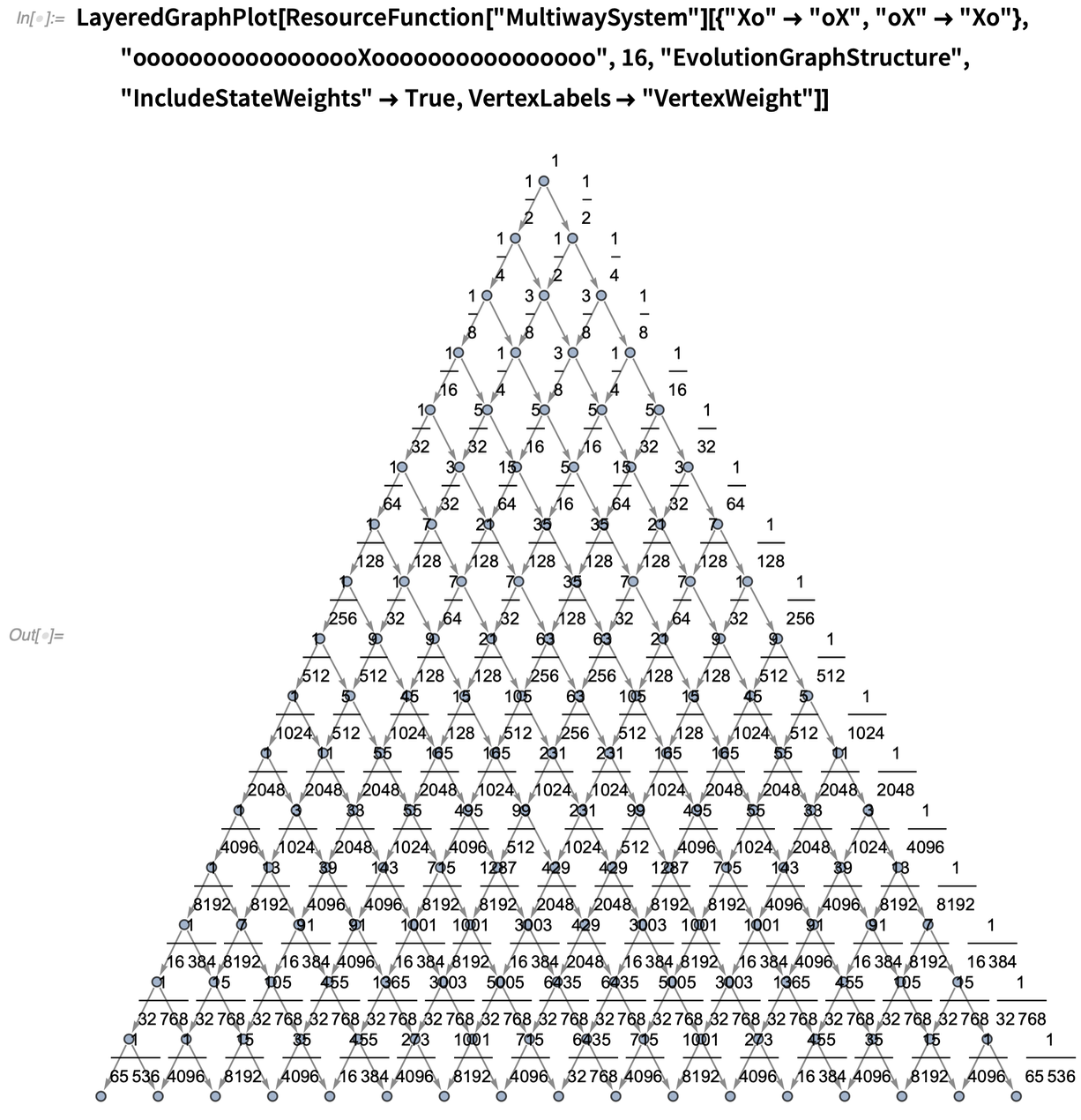✕ ```LayeredGraphPlot[ ResourceFunction["MultiwaySystem"][{"Xo" -> "oX", "oX" -> "Xo"}, "ooooooooooooooooXoooooooooooooooo", 16, "EvolutionGraphStructure", "IncludeStateWeights" -> True, VertexLabels -> "VertexWeight"]]```

now containing 17 distinct states at its final evolution step: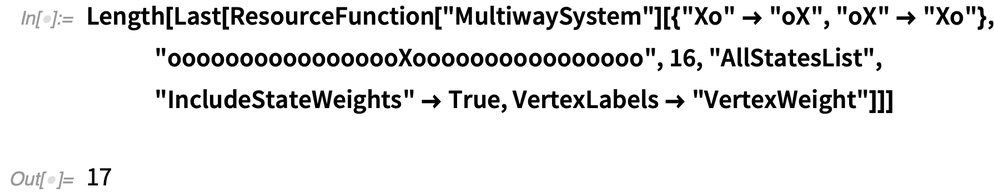✕ ```Length[Last[ ResourceFunction["MultiwaySystem"][{"Xo" -> "oX", "oX" -> "Xo"}, "ooooooooooooooooXoooooooooooooooo", 16, "AllStatesList", "IncludeStateWeights" -> True, VertexLabels -> "VertexWeight"]]]```

Once again, extracting the last half of the vertex weights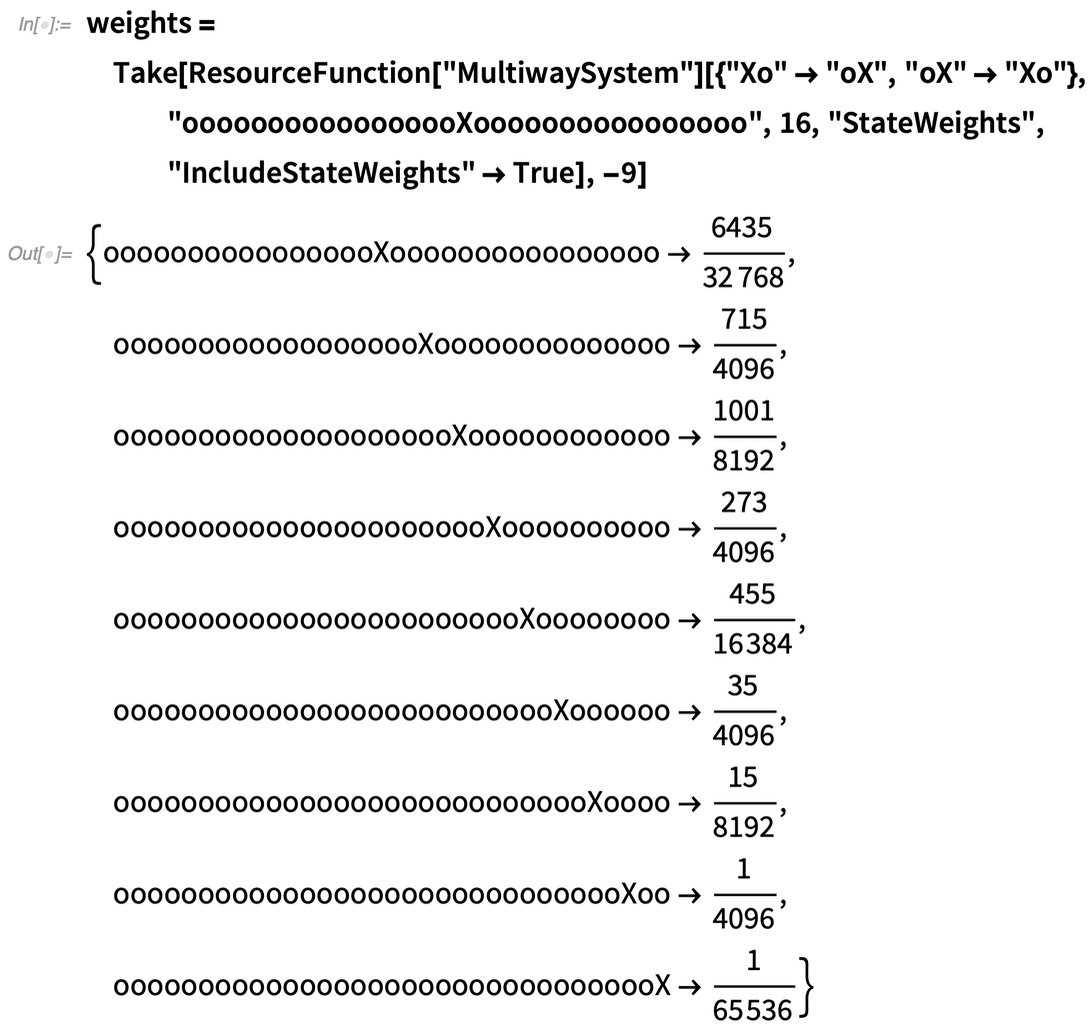✕ ```weights = Take[ResourceFunction["MultiwaySystem"][{"Xo" -> "oX", "oX" -> "Xo"}, "ooooooooooooooooXoooooooooooooooo", 16, "StateWeights", "IncludeStateWeights" -> True], -9]```

thus allows us to reconstruct the complete spatial intensity pattern on both sides as: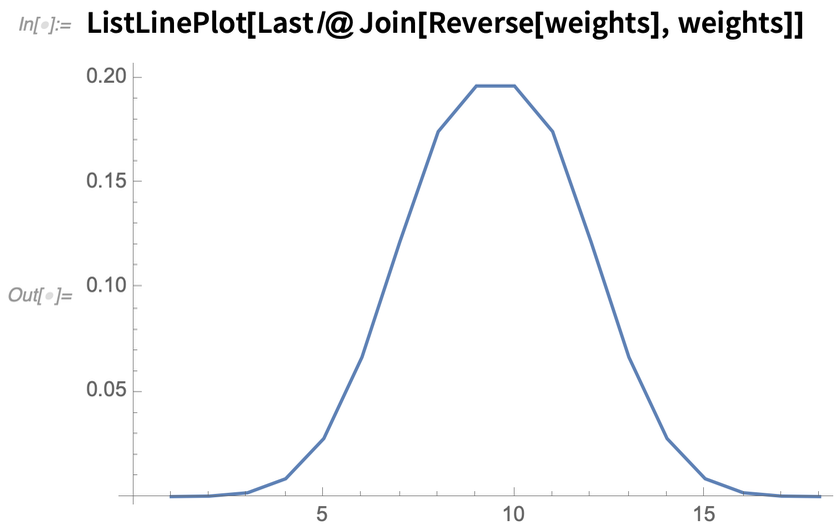✕ `ListLinePlot[Last /@ Join[Reverse[weights], weights]]`

Compare now against the expected single-slit photon diffraction pattern, as predicted by conventional quantum mechanics: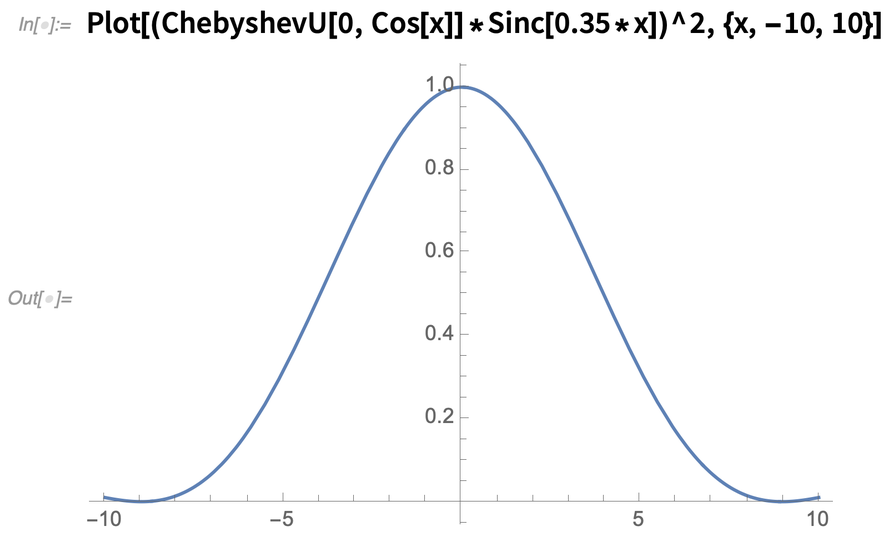✕ `Plot[(ChebyshevU[0, Cos[x]]*Sinc[0.35*x])^2, {x, -10, 10}]`

Our multiway approximation does indeed seem to be converging to the known analytical result! However, single-slit diffraction is a somewhat trivial phenomenon, since it lacks many of the destructive interference effects that make multi-slit diffraction phenomena so much more complex and interesting. In fact, its triviality can be witnessed explicitly by observing that its branchial graph (and, therefore, the associated projective Hilbert space that describes this particular quantum system) is strictly one-dimensional, reflecting the presence of only a single identifiable quantum subsystem: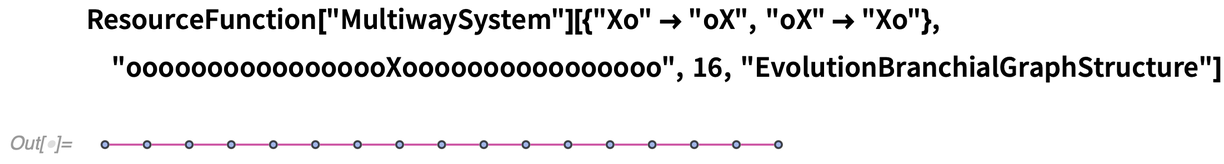✕ ```ResourceFunction["MultiwaySystem"][{"Xo" -> "oX", "oX" -> "Xo"}, "ooooooooooooooooXoooooooooooooooo", 16, \ "EvolutionBranchialGraphStructure"]```

So what happens if we try a two-slit diffraction case? We can do this very easily by just adding a second “X” (corresponding to a second photon propagating through a second slit) to our previous initial condition, with a small separation between the first and second slits, e.g. “oooooooooXoooXooooooooo”, thus yielding the following slightly more complicated evolution graph: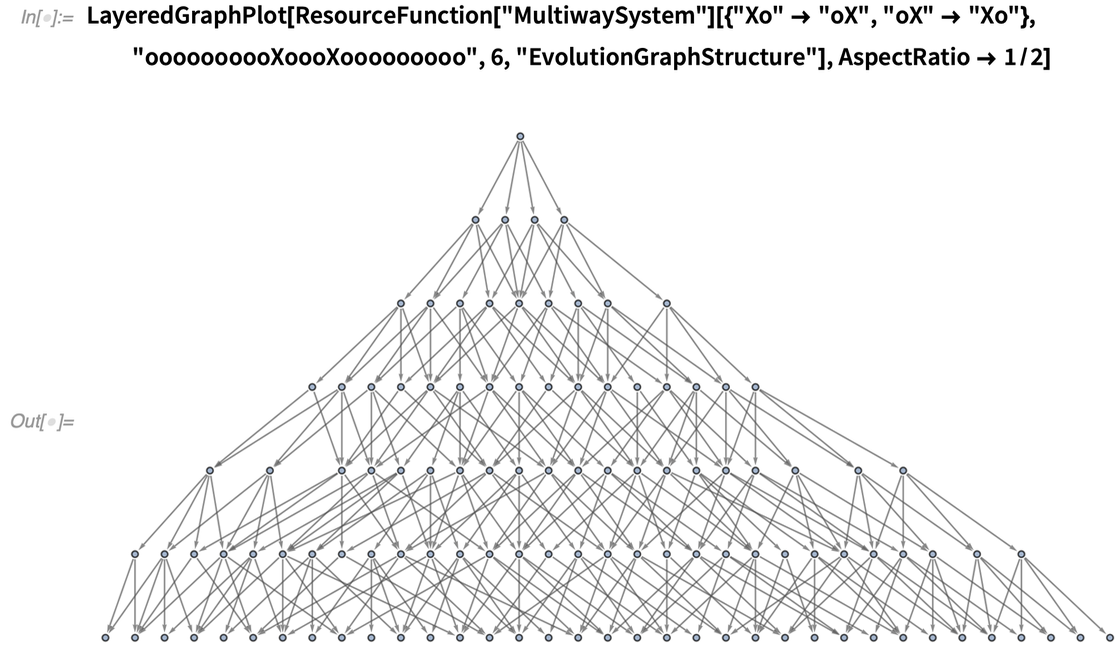✕ ```LayeredGraphPlot[ ResourceFunction["MultiwaySystem"][{"Xo" -> "oX", "oX" -> "Xo"}, "oooooooooXoooXooooooooo", 6, "EvolutionGraphStructure"], AspectRatio -> 1/2]```

There now exist 35 distinct states at the final evolution step: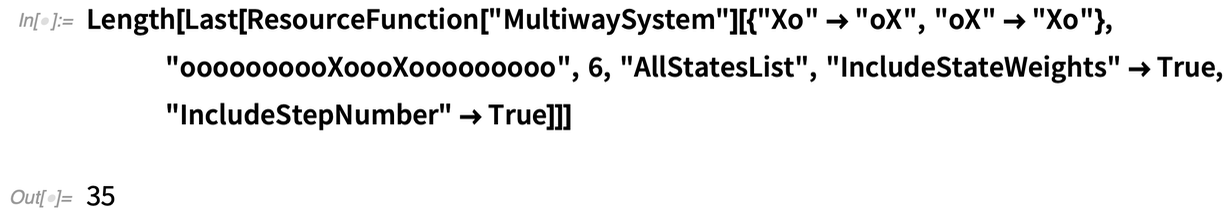✕ ```Length[Last[ ResourceFunction["MultiwaySystem"][{"Xo" -> "oX", "oX" -> "Xo"}, "oooooooooXoooXooooooooo", 6, "AllStatesList", "IncludeStateWeights" -> True, "IncludeStepNumber" -> True]]]```

So we extract the last half of the state weights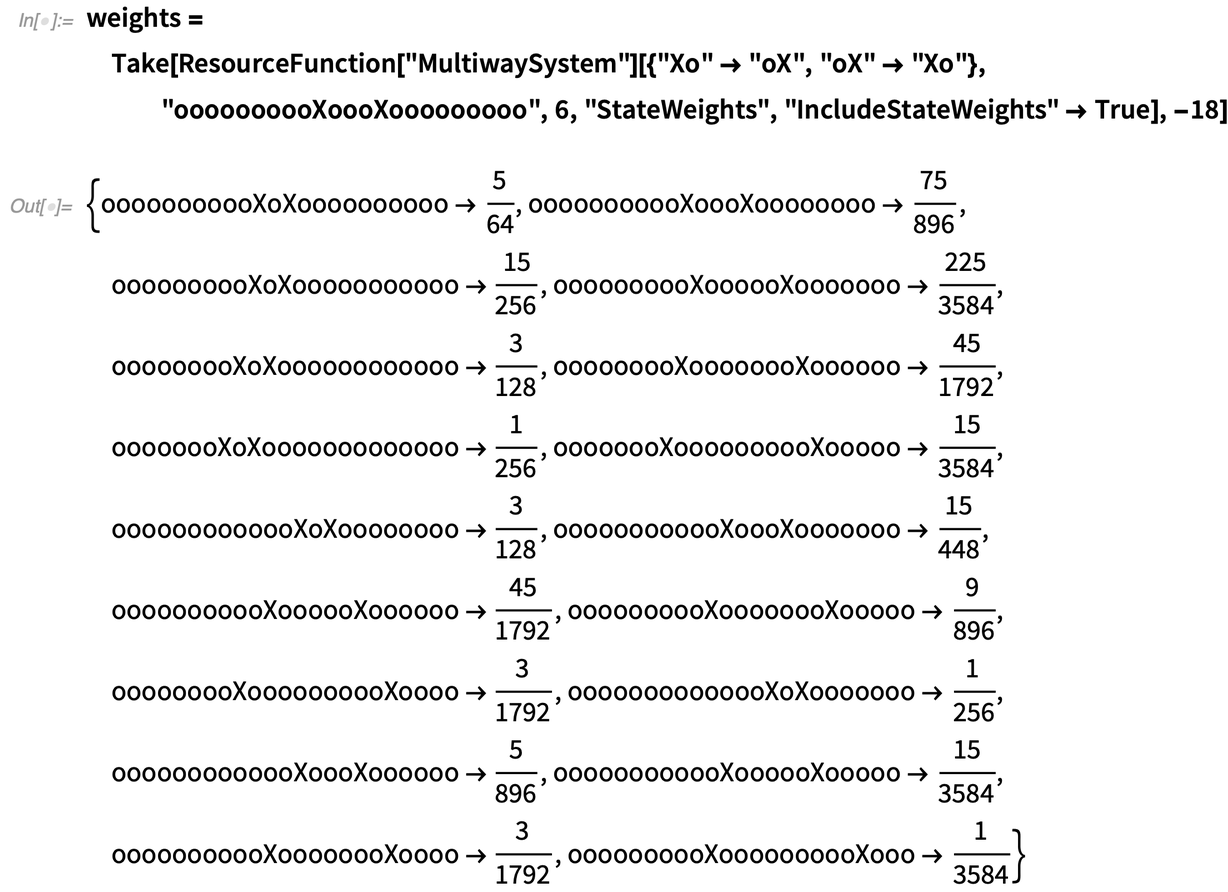✕ ```weights = Take[ResourceFunction["MultiwaySystem"][{"Xo" -> "oX", "oX" -> "Xo"}, "oooooooooXoooXooooooooo", 6, "StateWeights", "IncludeStateWeights" -> True], -18]```

and reconstruct the spatial intensity pattern in the usual way: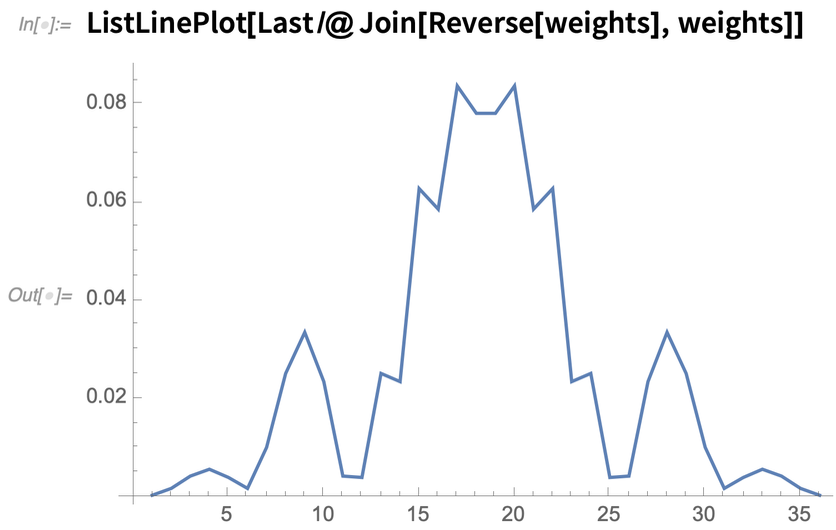✕ `ListLinePlot[Last /@ Join[Reverse[weights], weights]]`

Okay, now something pretty interesting is starting to happen! We see one large central maximum (as one might expect, given the previous case), but now the maximum has two immediate minima on either side of it, followed by smaller fringe maxima on either side of those etc. Let’s repeat this analysis by running the multiway system for a few more evolution steps, to see what this new pattern might be converging to: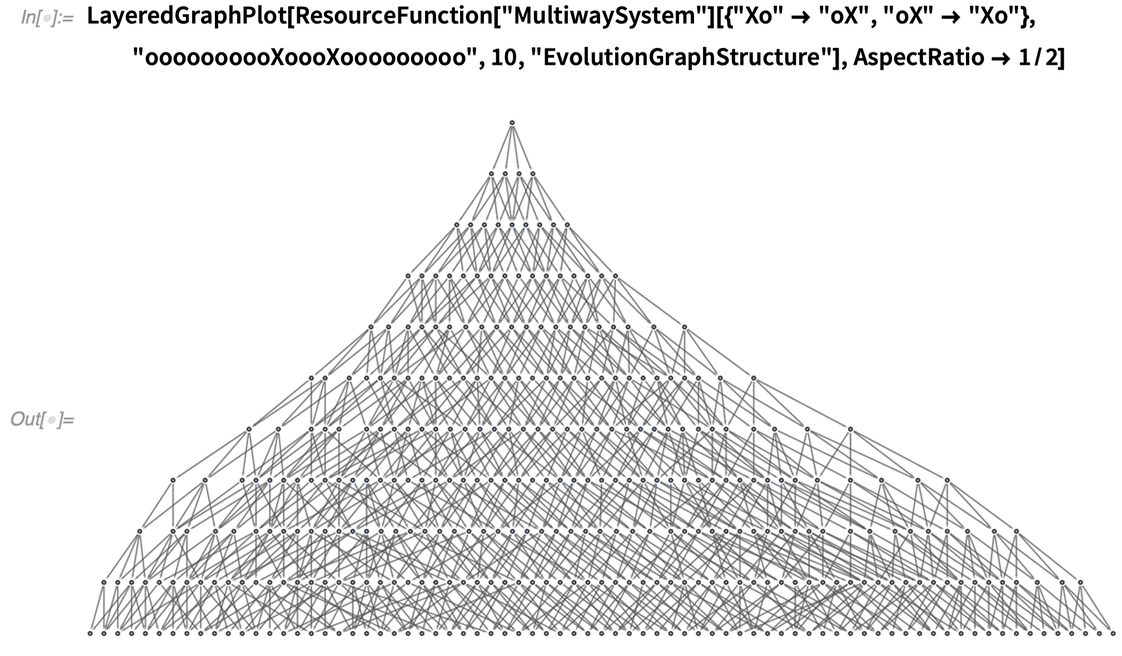✕ ```LayeredGraphPlot[ ResourceFunction["MultiwaySystem"][{"Xo" -> "oX", "oX" -> "Xo"}, "oooooooooXoooXooooooooo", 10, "EvolutionGraphStructure"], AspectRatio -> 1/2]```

Now there are 75 distinct states at the final step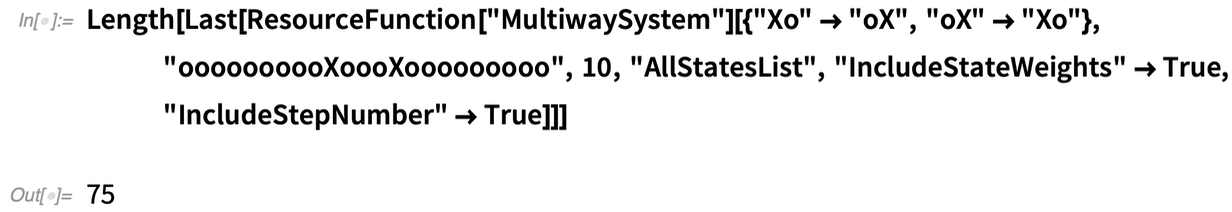✕ ```Length[Last[ ResourceFunction["MultiwaySystem"][{"Xo" -> "oX", "oX" -> "Xo"}, "oooooooooXoooXooooooooo", 10, "AllStatesList", "IncludeStateWeights" -> True, "IncludeStepNumber" -> True]]]```

so by extracting the last half of the state weights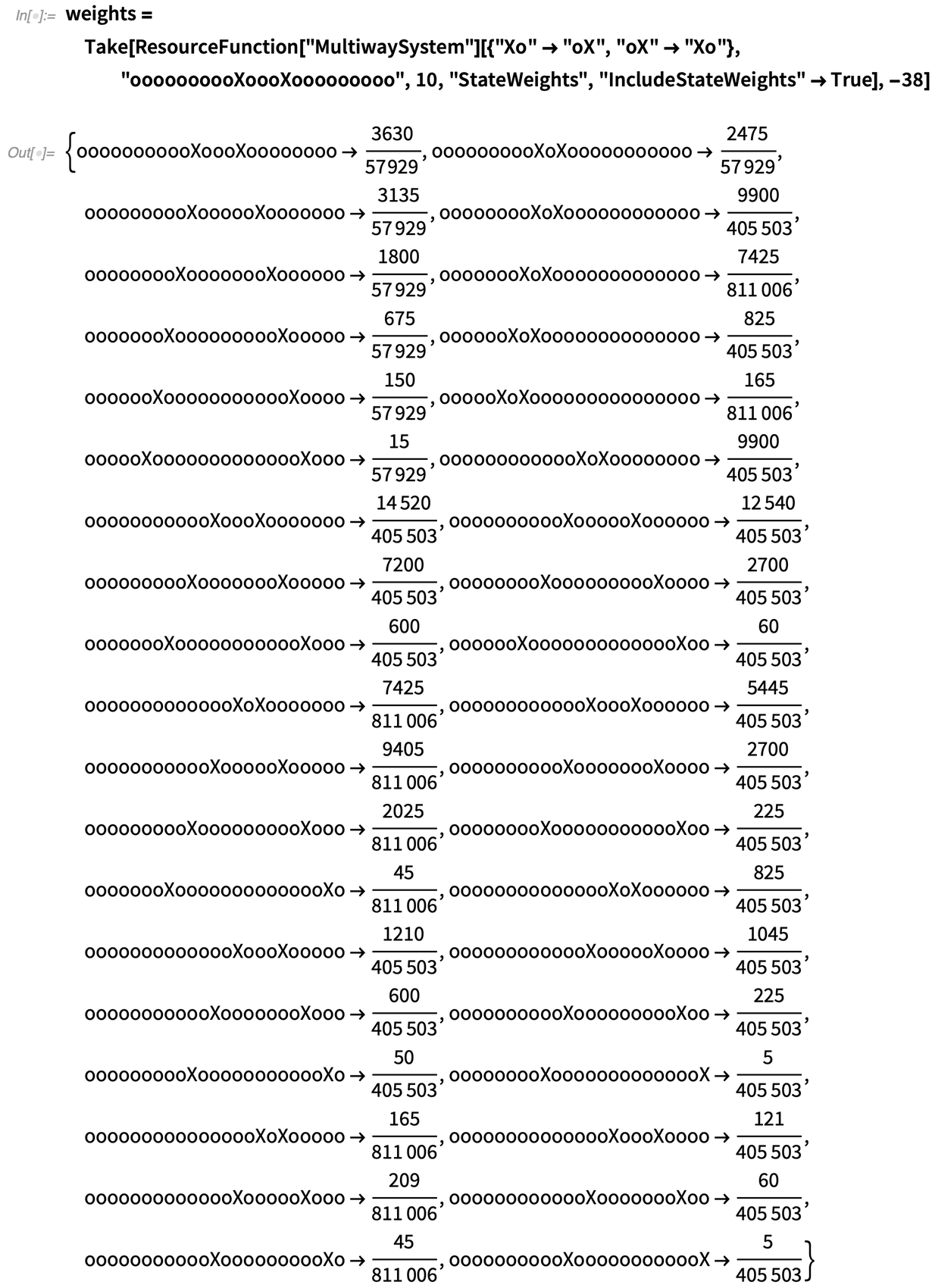✕ ```weights = Take[ResourceFunction["MultiwaySystem"][{"Xo" -> "oX", "oX" -> "Xo"}, "oooooooooXoooXooooooooo", 10, "StateWeights", "IncludeStateWeights" -> True], -38]```

we can reconstruct the following higher-resolution spatial intensity pattern: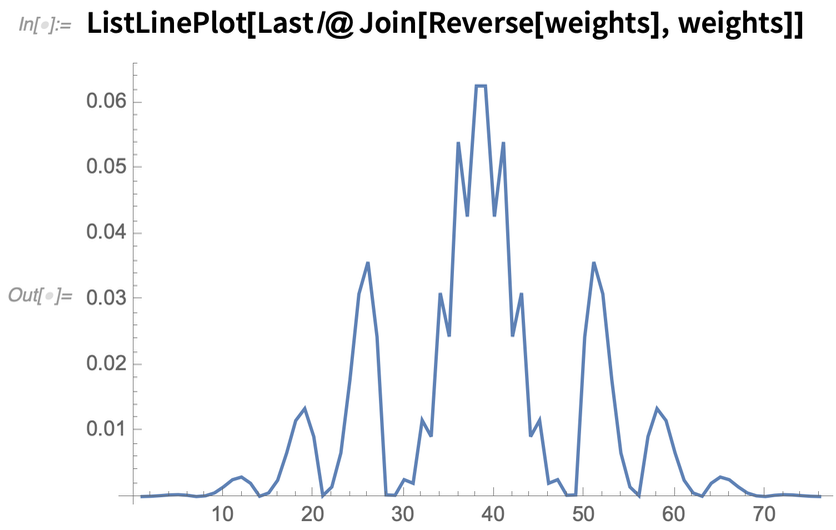✕ `ListLinePlot[Last /@ Join[Reverse[weights], weights]]`

Comparing once more against the expected double-slit photon diffraction pattern from conventional quantum mechanics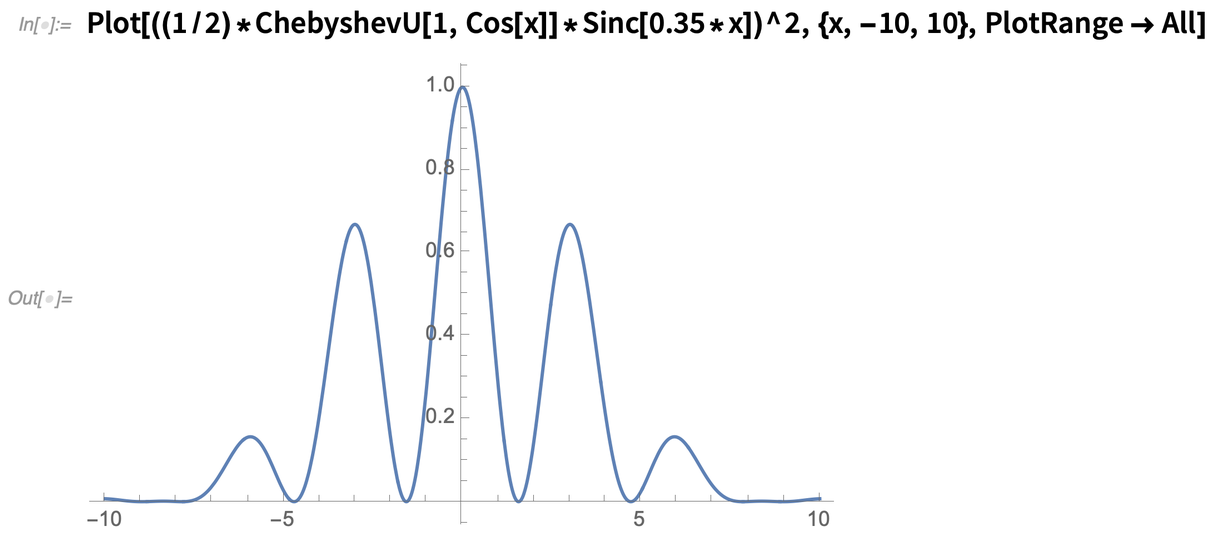✕ ```Plot[((1/2)*ChebyshevU[1, Cos[x]]*Sinc[0.35*x])^2, {x, -10, 10}, PlotRange -> All]```

we see astonishingly good convergence to the analytical result, with correct prediction of the relative positions of the minima and the fringe maxima, as well as of the relative intensities of the interference fringes (compared both to the central maximum and to each other) etc. Since there are two interacting quantum subsystems in this second case (one associated with each slit), the branchial graph, and hence the associated projective Hilbert space, is now two-dimensional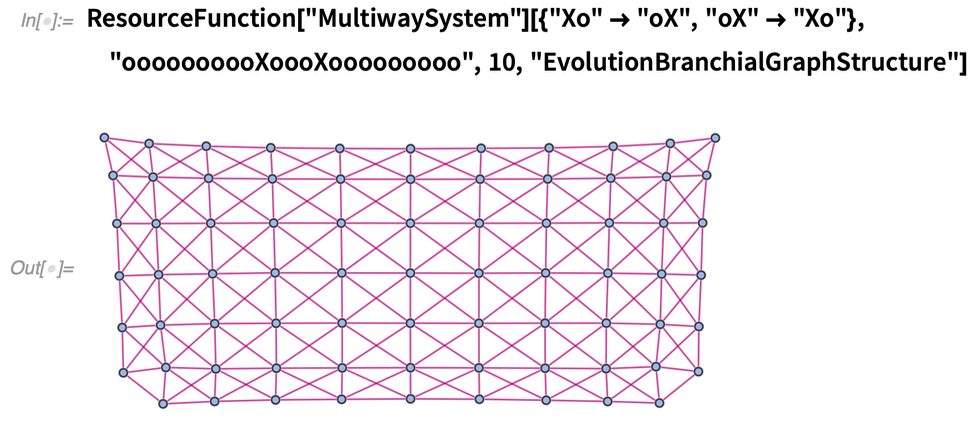✕ ```ResourceFunction["MultiwaySystem"][{"Xo" -> "oX", "oX" -> "Xo"}, "oooooooooXoooXooooooooo", 10, \ "EvolutionBranchialGraphStructure"]```

where the “rounding off” at the corners of the branchial graph is a simple boundary effect associated with the finite nature of the string.

Finally, just to confirm the robustness of these correspondences, let us consider the three-slit diffraction case, with an example initial state “oooooooXXXooooooo”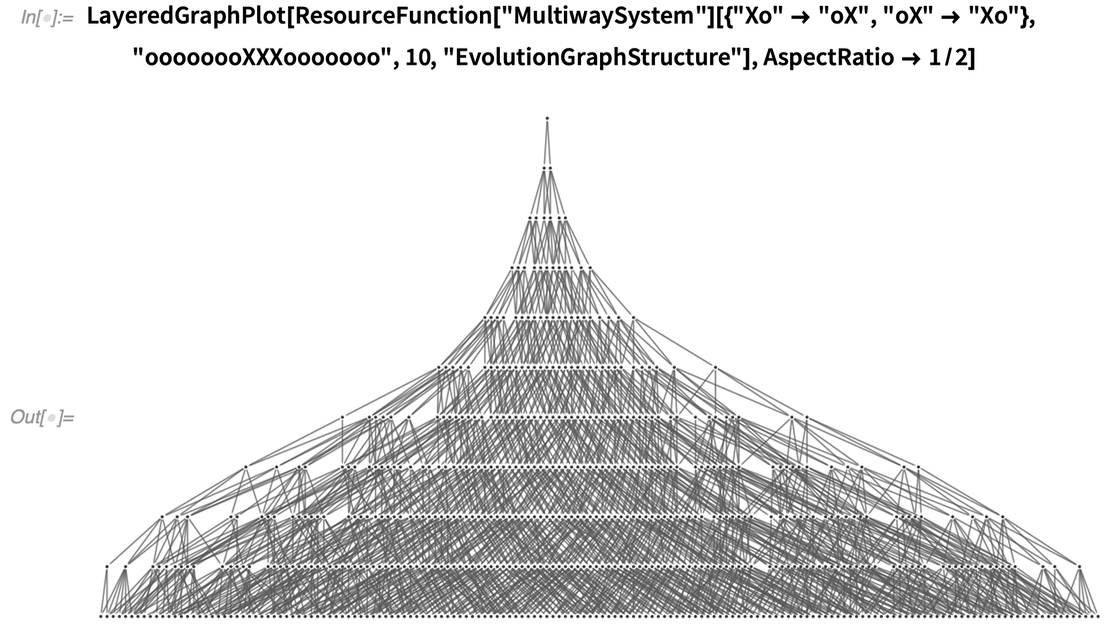✕ ```LayeredGraphPlot[ ResourceFunction["MultiwaySystem"][{"Xo" -> "oX", "oX" -> "Xo"}, "oooooooXXXooooooo", 10, "EvolutionGraphStructure"], AspectRatio -> 1/2]```

yielding 162 distinct states at the final evolution step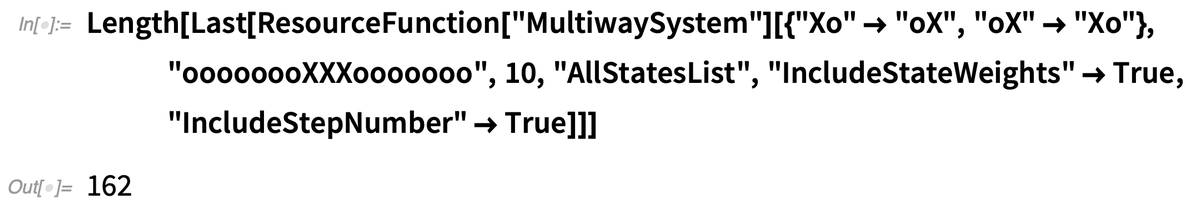✕ ```Length[Last[ ResourceFunction["MultiwaySystem"][{"Xo" -> "oX", "oX" -> "Xo"}, "oooooooXXXooooooo", 10, "AllStatesList", "IncludeStateWeights" -> True, "IncludeStepNumber" -> True]]]```

with corresponding weights for the right-hand side of the “screen”: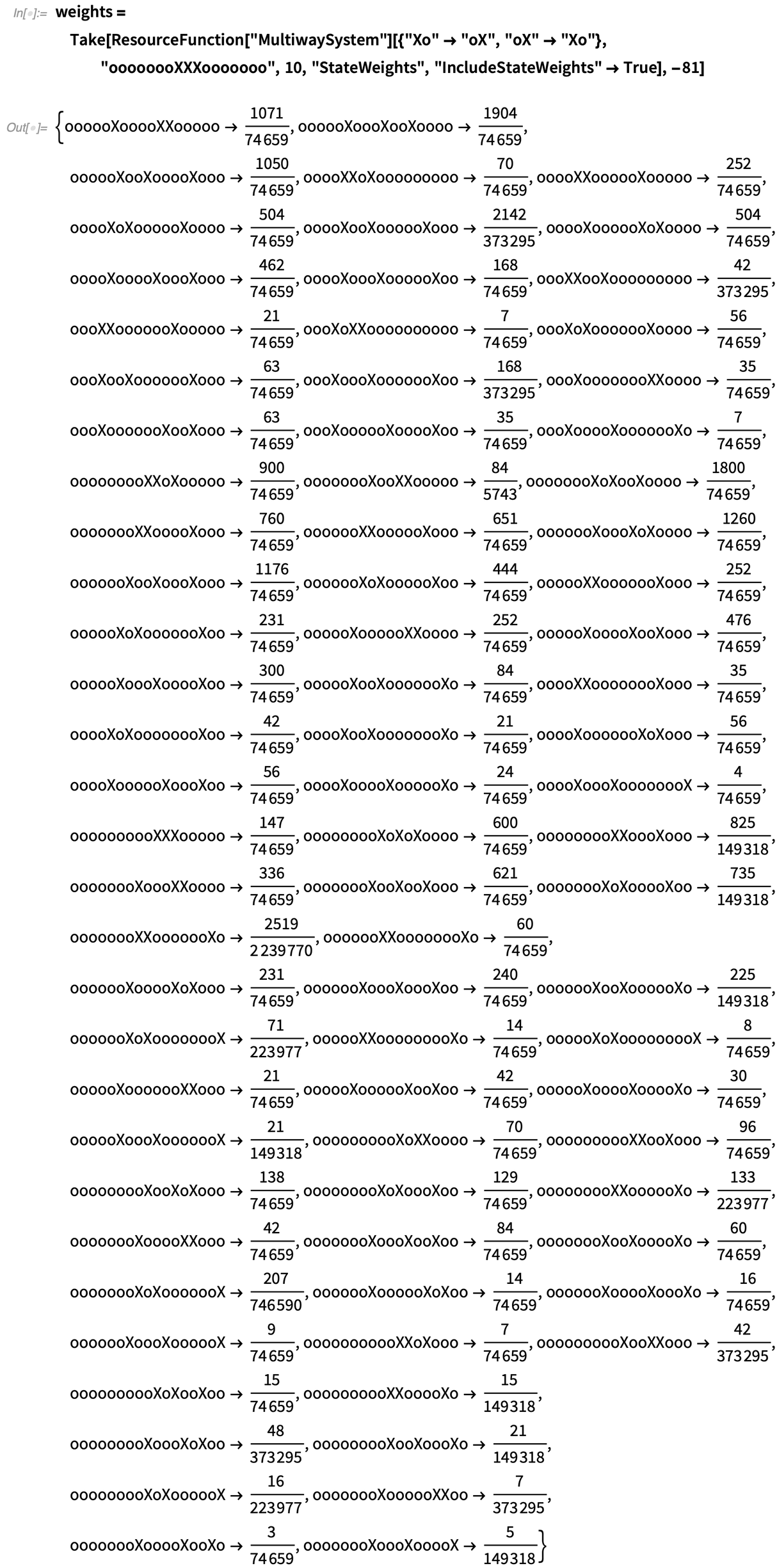✕ ```weights = Take[ResourceFunction["MultiwaySystem"][{"Xo" -> "oX", "oX" -> "Xo"}, "oooooooXXXooooooo", 10, "StateWeights", "IncludeStateWeights" -> True], -81]```

This results in the final spatial intensity pattern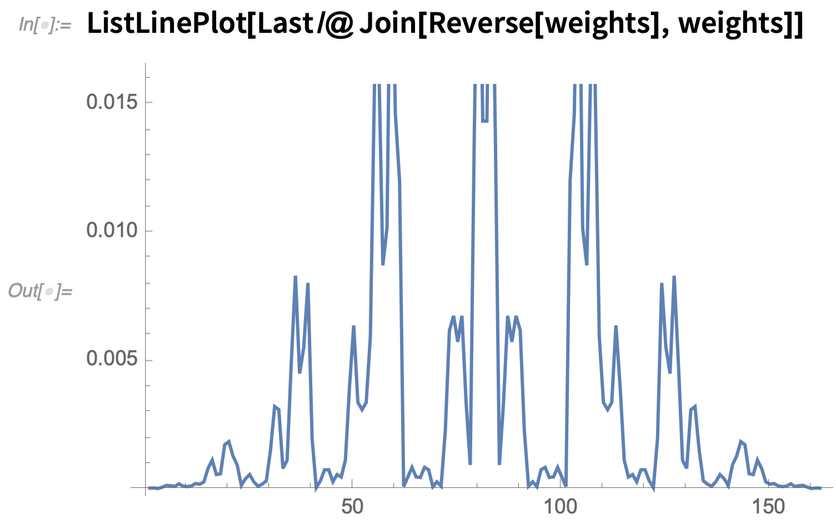✕ `ListLinePlot[Last /@ Join[Reverse[weights], weights]]`

which again compares exceptionally favorably (particularly given the relatively small string size and low number of evolution steps) to the triple-slit diffraction pattern predicted from the usual Fresnel integral approximation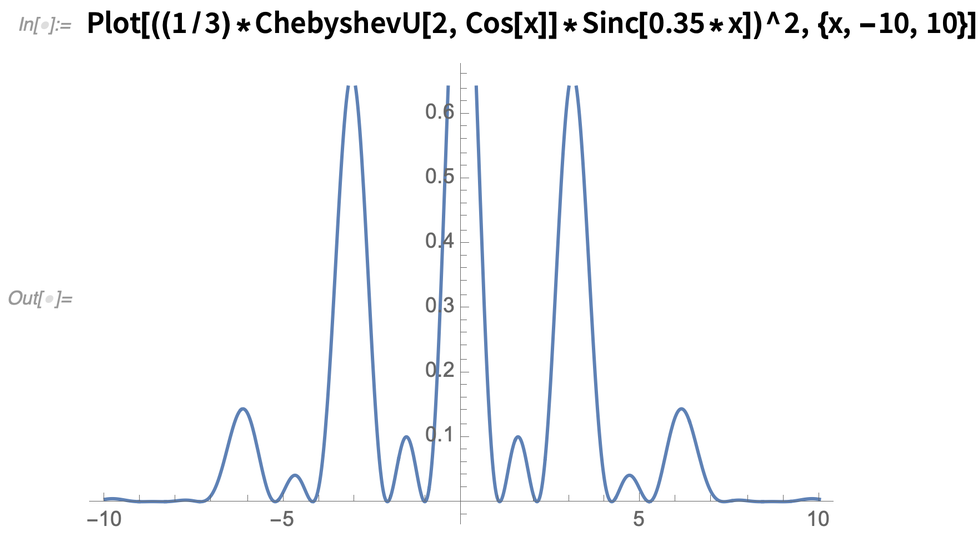✕ `Plot[((1/3)*ChebyshevU[2, Cos[x]]*Sinc[0.35*x])^2, {x, -10, 10}]`

with the associated branchial graph now corresponding, unsurprisingly, to a fully three-dimensional projective Hilbert space: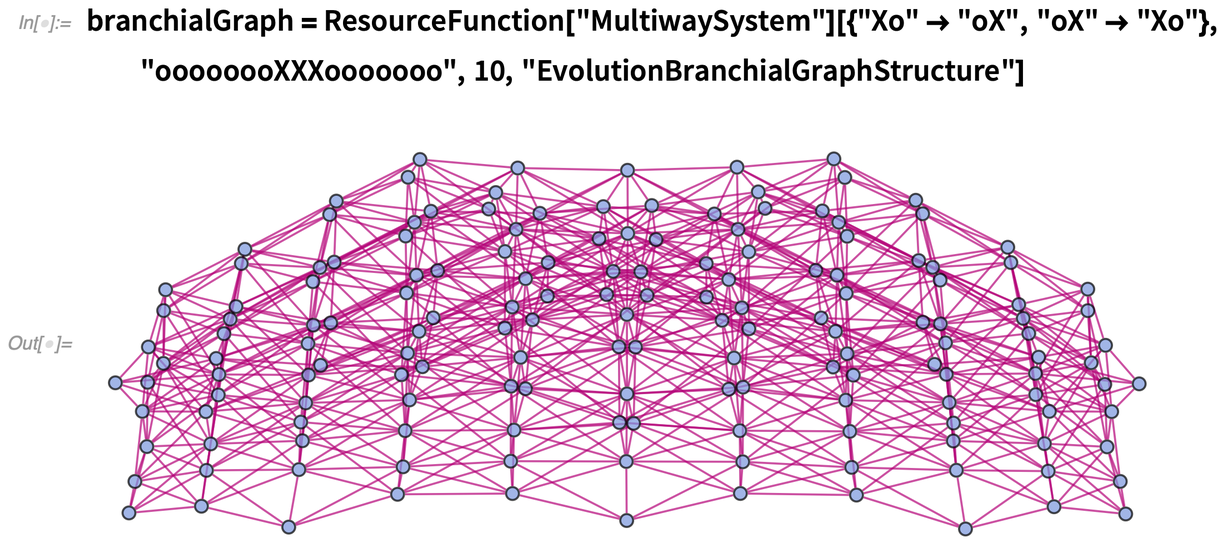✕ ```branchialGraph = ResourceFunction["MultiwaySystem"][{"Xo" -> "oX", "oX" -> "Xo"}, "oooooooXXXooooooo", 10, "EvolutionBranchialGraphStructure"]```

Therefore it really does seem that, with these very simple string multiway system models of quantum mechanics, we’ve been able to capture both the key qualitative and quantitative aspects of the double-slit experiment, and of quantum interference phenomena more generally, exactly as my formulation originally predicted. But how does any of this actually work? What’s really going on underneath? Ultimately, it’s just a simple theorem of combinatorics (with a smattering of elementary number theory), but understanding intuitively why this theorem holds requires first delving into some of the gory details of position bases, completion procedures, induced causal invariance and the relationship between branchial geometry and quantum phase….

## How It All Works: Completion Procedures, Phase Changes and the Combinatorics of Destructive Interference

First of all, let me attempt to demonstrate explicitly how the construction of the string position basis works in the particular case of the double-slit setup I described in the last section. I should stress that the scheme I describe here for encoding the position basis in terms of substring positions is only one possible such method, and doubtless it can be improved in many ways. The essential idea here is to encode which “region” contains the photon using the sum of the “X” substring positions, and then to encode precisely where the photon is “within” that region using the difference of those same positions.

For instance, consider the simple two-slit case described previously, defined by an initial condition “ooooooooXXoooooooo”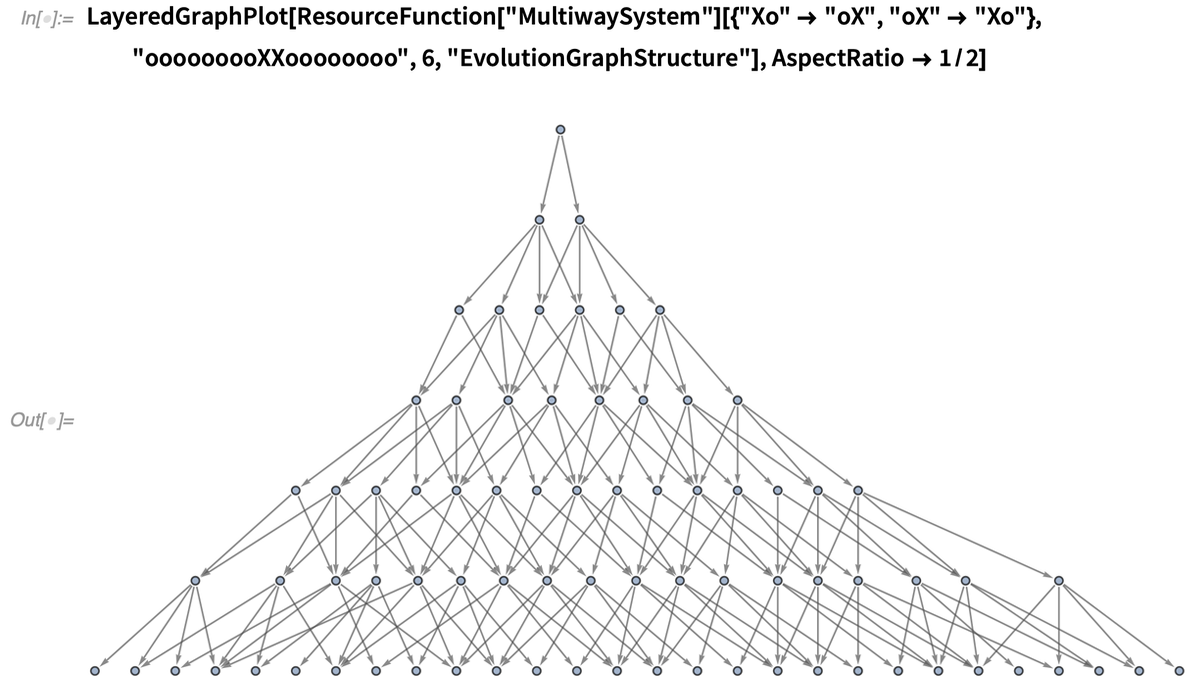✕ ```LayeredGraphPlot[ ResourceFunction["MultiwaySystem"][{"Xo" -> "oX", "oX" -> "Xo"}, "ooooooooXXoooooooo", 6, "EvolutionGraphStructure"], AspectRatio -> 1/2]```

with 28 distinct states at the final step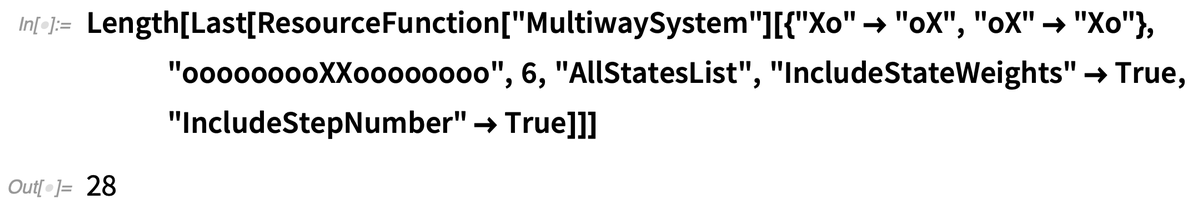✕ ```Length[Last[ ResourceFunction["MultiwaySystem"][{"Xo" -> "oX", "oX" -> "Xo"}, "ooooooooXXoooooooo", 6, "AllStatesList", "IncludeStateWeights" -> True, "IncludeStepNumber" -> True]]]```

and consider, in particular, the right-hand side of our fictional “experimental apparatus”, characterized by the following 14 states (shown here in the standard order, as sorted internally by "StateWeights"):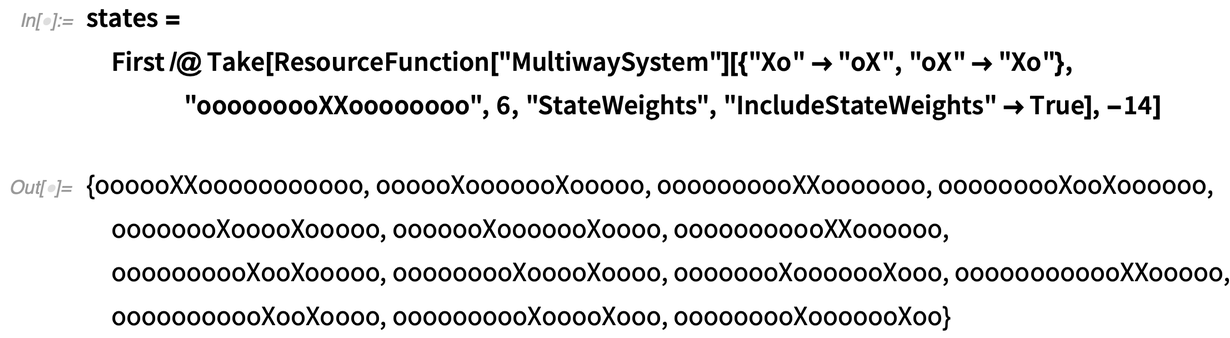✕ ```states = First /@ Take[ResourceFunction["MultiwaySystem"][{"Xo" -> "oX", "oX" -> "Xo"}, "ooooooooXXoooooooo", 6, "StateWeights", "IncludeStateWeights" -> True], -14]```

In our fictional experimental setup, there exist three distinct spatial regions: a central separator region, a region corresponding to the right-hand slit and a further right-hand separator region. Which of the three spatial regions the photon is contained within is then encoded by the sum of the string positions of the individual “X”s, i.e.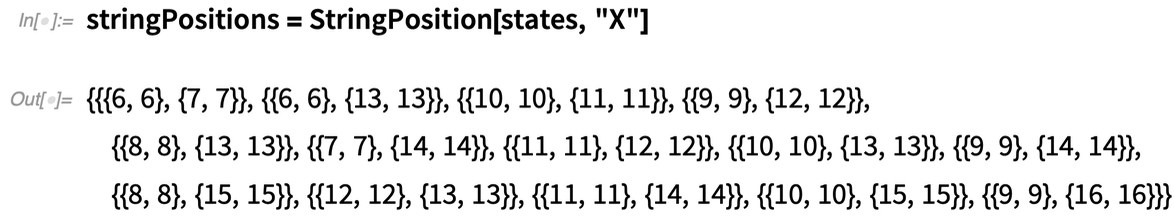✕ `stringPositions = StringPosition[states, "X"]`

where, in this particular case, the sum corresponds to either 21, 23 or 25 (ignoring the 13 and the 19 at the beginning of the following list, which correspond to boundary cutoff effects resulting from the finiteness of the string):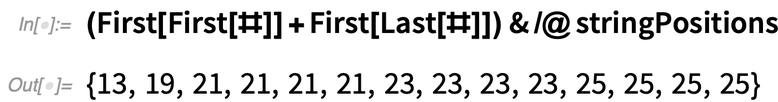✕ `(First[First[#]] + First[Last[#]]) & /@ stringPositions`

The position of the photon within that region is then encoded by the difference in the positions of the two “X”s, which here correspond to either 1, 3, 5 or 7: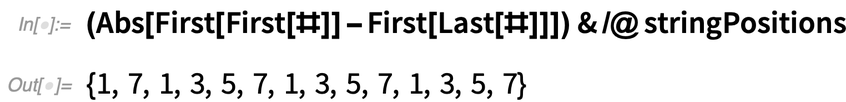✕ `(Abs[First[First[#]] - First[Last[#]]]) & /@ stringPositions`

Thanks to this elegant encoding of the position basis, it is therefore guaranteed that, by looking at the last half of the sequence of weights (as previously indicated), these weights will necessarily correspond to the intensities of incident photons at monotonically increasing positions on the fictional experimental “screen”, starting from the center and ending at the far right, exactly as required.

Okay, so that takes care of the mapping between the positions of microstates in branchial space and the corresponding spatial positions of the photons on the “screen”, but how do the crucial concepts of quantum phase and interference make themselves manifest within this particular model of double-slit diffraction? To understand this, we begin by noticing that our simulated “Huygens–Fresnel” rules are trivially causal invariant: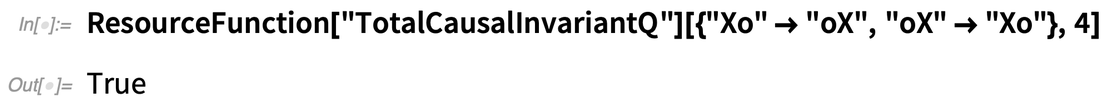✕ ```ResourceFunction["TotalCausalInvariantQ"][{"Xo" -> "oX", "oX" -> "Xo"}, 4]```

And, as detailed in my quantum mechanics paper, all causal invariant multiway systems may be considered to have been “derived” by an appropriate application of a Knuth–Bendix completion procedure from a previously “uncompleted” non-causal invariant system, by a process that is directly analogous to wavefunction collapse in the standard Copenhagen interpretation of quantum mechanics. For instance, starting from the following simple multiway system consisting of two independent evolution branches (which we can think of as corresponding to two independent paths of history in which the photon either goes through one slit or goes through the other, in the case of a minimal two-slit diffraction experiment)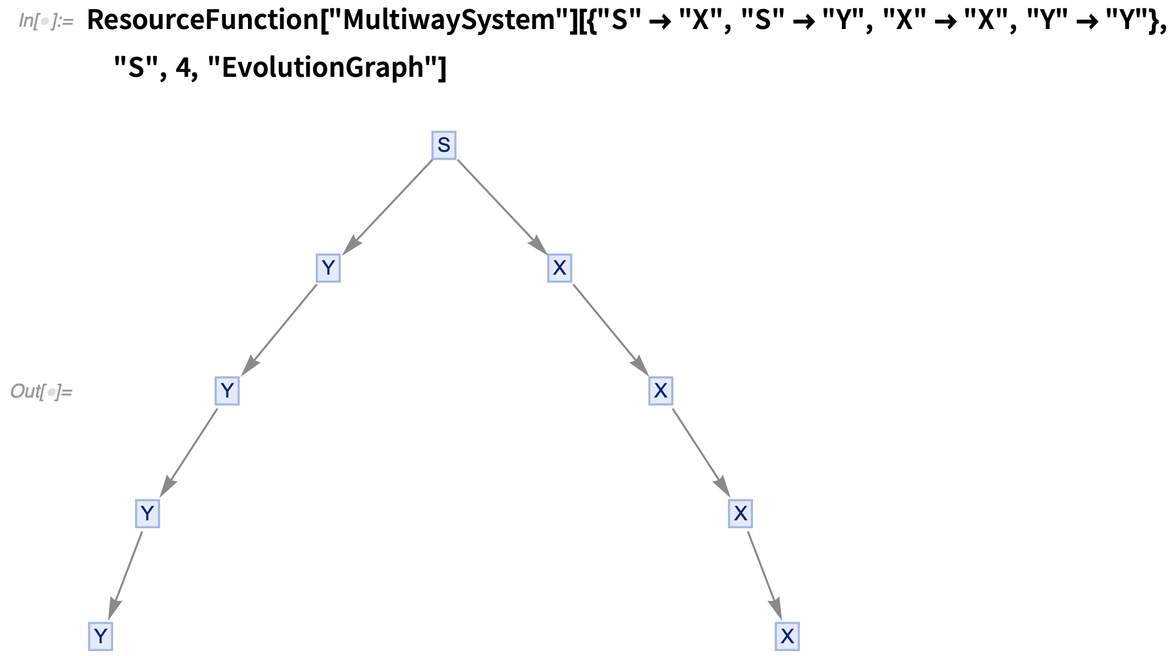✕ ```ResourceFunction["MultiwaySystem"][{"S" -> "X", "S" -> "Y", "X" -> "X", "Y" -> "Y"}, "S", 4, "EvolutionGraph"]```

we can see that this also constitutes a minimal model for a non-causal invariant multiway system (in which there exists a single branch pair that is guaranteed never to reconverge):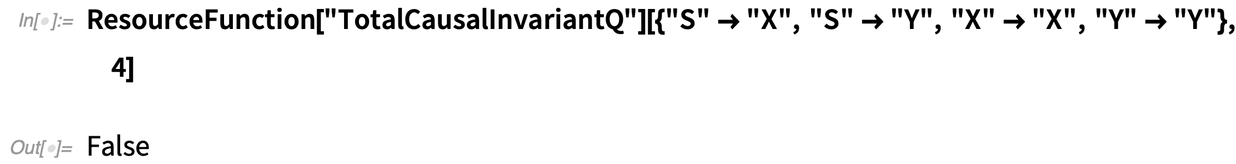✕ ```ResourceFunction["TotalCausalInvariantQ"][{"S" -> "X", "S" -> "Y", "X" -> "X", "Y" -> "Y"}, 4]```

However, we can force these two non-intersecting branches to interact (and hence to collapse down to a single effective branch of history) by performing a Knuth–Bendix completion in the standard fashion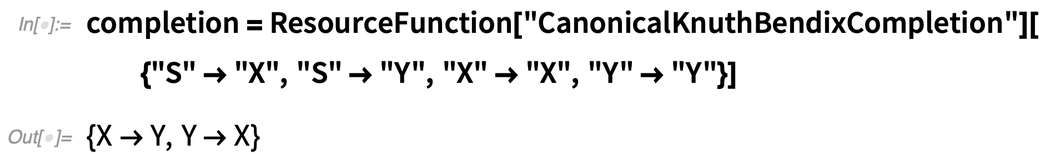✕ ```completion = ResourceFunction["CanonicalKnuthBendixCompletion"][{"S" -> "X", "S" -> "Y", "X" -> "X", "Y" -> "Y"}]```

thus obtaining the new evolution history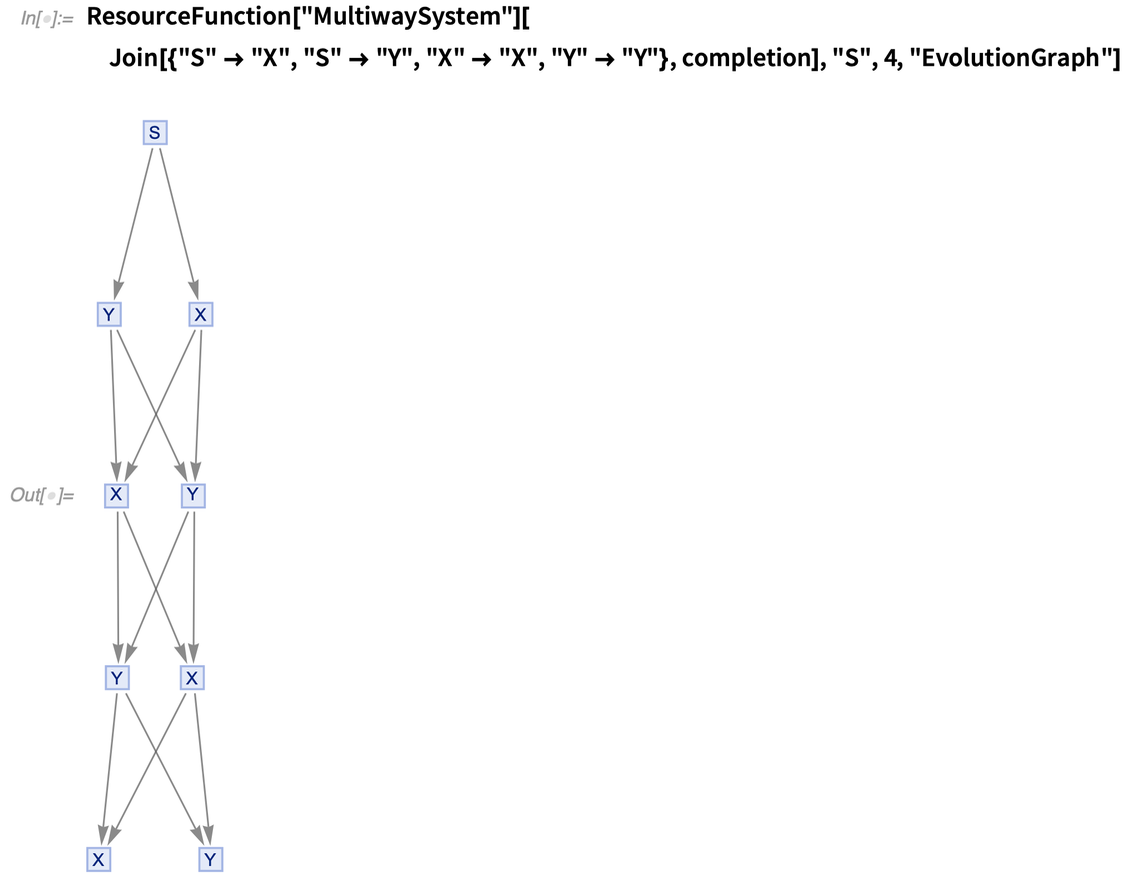✕ ```ResourceFunction["MultiwaySystem"][ Join[{"S" -> "X", "S" -> "Y", "X" -> "X", "Y" -> "Y"}, completion], "S", 4, "EvolutionGraph"]```

which is now trivially causal invariant: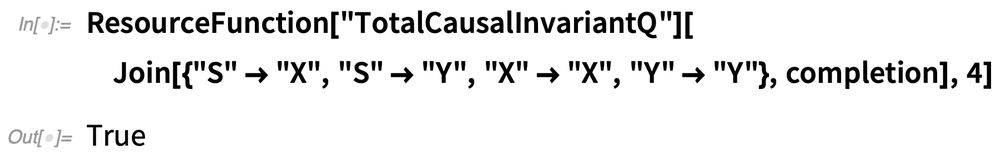✕ ```ResourceFunction["TotalCausalInvariantQ"][ Join[{"S" -> "X", "S" -> "Y", "X" -> "X", "Y" -> "Y"}, completion], 4]```

So how does a completion procedure achieve the destructive interference effects that are so crucial for reproducing the results of the double-slit experiment just shown? As I described in my quantum mechanics paper, the basic idea is to think of the phase difference between two paths in the multiway system as corresponding to the ratio of branchlike- to spacelike-separated events along those paths (or, strictly speaking, to twice the ArcTan of that ratio). Thus, if you have two paths along which every pair of events is purely spacelike separated (corresponding to a pair of isomorphic paths in the multiway system), then the phase difference between the two paths is exactly zero. This implies that the two paths merge and the corresponding state weights add, and so one ultimately achieves perfect constructive interference. If you have two paths along which every pair of events is purely branchlike separated (corresponding to a pair of non-intersecting paths in the multiway system, as seen in the first example), then the phase difference between the two paths is exactly π, meaning that they perfectly destructively interfere.

But how does that destructive interference actually occur? Well, it’s entirely a consequence of the combinatorial structure of the completion procedure. Consider the element “X” within the pair of strings “Xo” and “oX”; in this particular case, the two “X”s are purely spacelike separated across the two states (since they occur in non-conflicting parts of the string), which means that the pair of states can be successfully completed to yield the common state “XX”, and no information is lost (i.e. one has perfect constructive interference). Consider now the element “X” within a pair of strings “Xo” and “XO”; in this new case, the two “X”s are purely branchlike separated across the two states (since they now occur in conflicting parts of the string, i.e. both at position 1), which means that the only consistent completion procedure for the two states will necessarily destroy any information about whether there was an “o” or an “O” at position 2. In other words, any completion procedure will necessarily yield a common state of the form "X"<>{"O","o"}, where the {...} here denotes an equivalence class of substrings, indicating that the information about whether the previous state contained an “o” or an “O” in at position 2 is now lost (i.e. one has perfect destructive interference).

To see the connection among phase difference, destructive interference and completion procedures more explicitly, it is helpful to consider a minimal illustrative example of a quantum harmonic oscillator, simulated within a simple string multiway system, using a scheme proposed as part of Patrick Geraghty’s Summer School project. Using the pair of rules “Xo”  “oX” and “oY”  “Yo”, we can use the character “X” to simulate a particle traveling from left to right and the character “Y” to simulate the same particle traveling back from right to left. Then we can use the character “O” to denote the boundaries of some finite-sized region of configuration space, with the pair of rules “XO”  “YO” and “OY”  “OX” thus effectively implementing reflective boundary conditions for the oscillating particle. As a consequence, we can change the period of the oscillator by simply modifying the length of the initial string, as shown here (for the case of oscillators corresponding to periods of 4, 6, 8 and 10, respectively)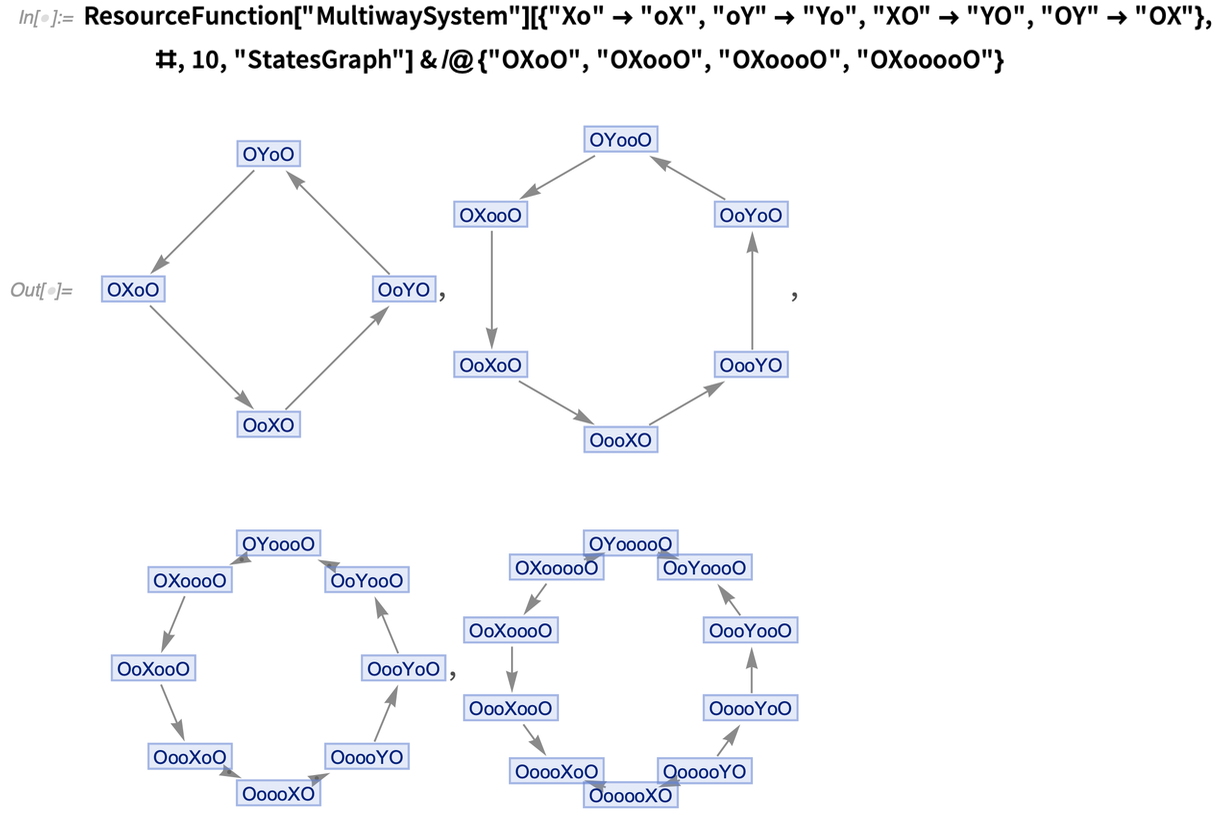✕ ```ResourceFunction["MultiwaySystem"][{"Xo" -> "oX", "oY" -> "Yo", "XO" -> "YO", "OY" -> "OX"}, #, 10, "StatesGraph"] & /@ {"OXoO", "OXooO", "OXoooO", "OXooooO"}```

with the associated evolution causal graphs: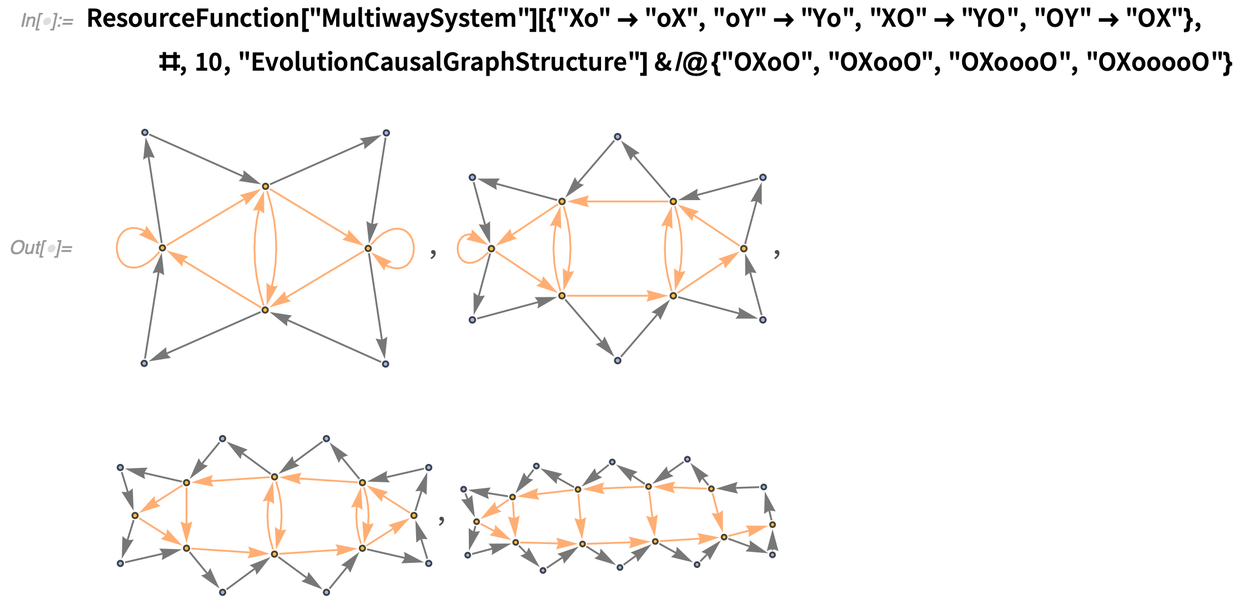✕ ```ResourceFunction["MultiwaySystem"][{"Xo" -> "oX", "oY" -> "Yo", "XO" -> "YO", "OY" -> "OX"}, #, 10, "EvolutionCausalGraphStructure"] & /@ {"OXoO", "OXooO", "OXoooO", "OXooooO"}```

For instance, here is a multiway system consisting of two independent branches—one containing a period-4 oscillator and the other containing a period-6 oscillator: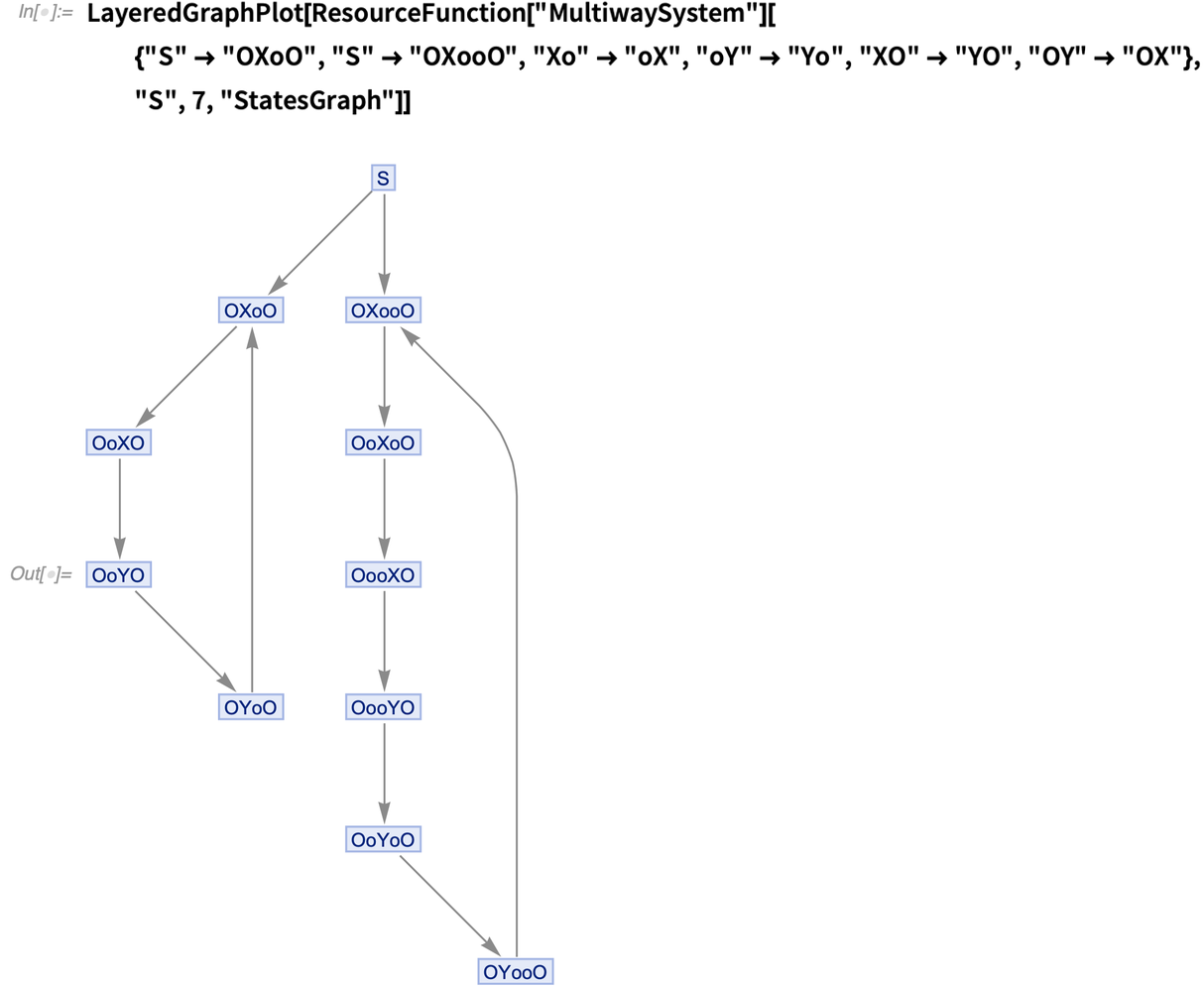✕ ```LayeredGraphPlot[ ResourceFunction["MultiwaySystem"][{"S" -> "OXoO", "S" -> "OXooO", "Xo" -> "oX", "oY" -> "Yo", "XO" -> "YO", "OY" -> "OX"}, "S", 7, "StatesGraph"]]```

However, though it is no doubt useful for pedagogical purposes, in practice we don’t actually need all of the “internal structure” of a simulated particle bouncing between two simulated walls; we can just generate the exact same form of a cyclic multiway system using purely single-character rewrite cycles as follows: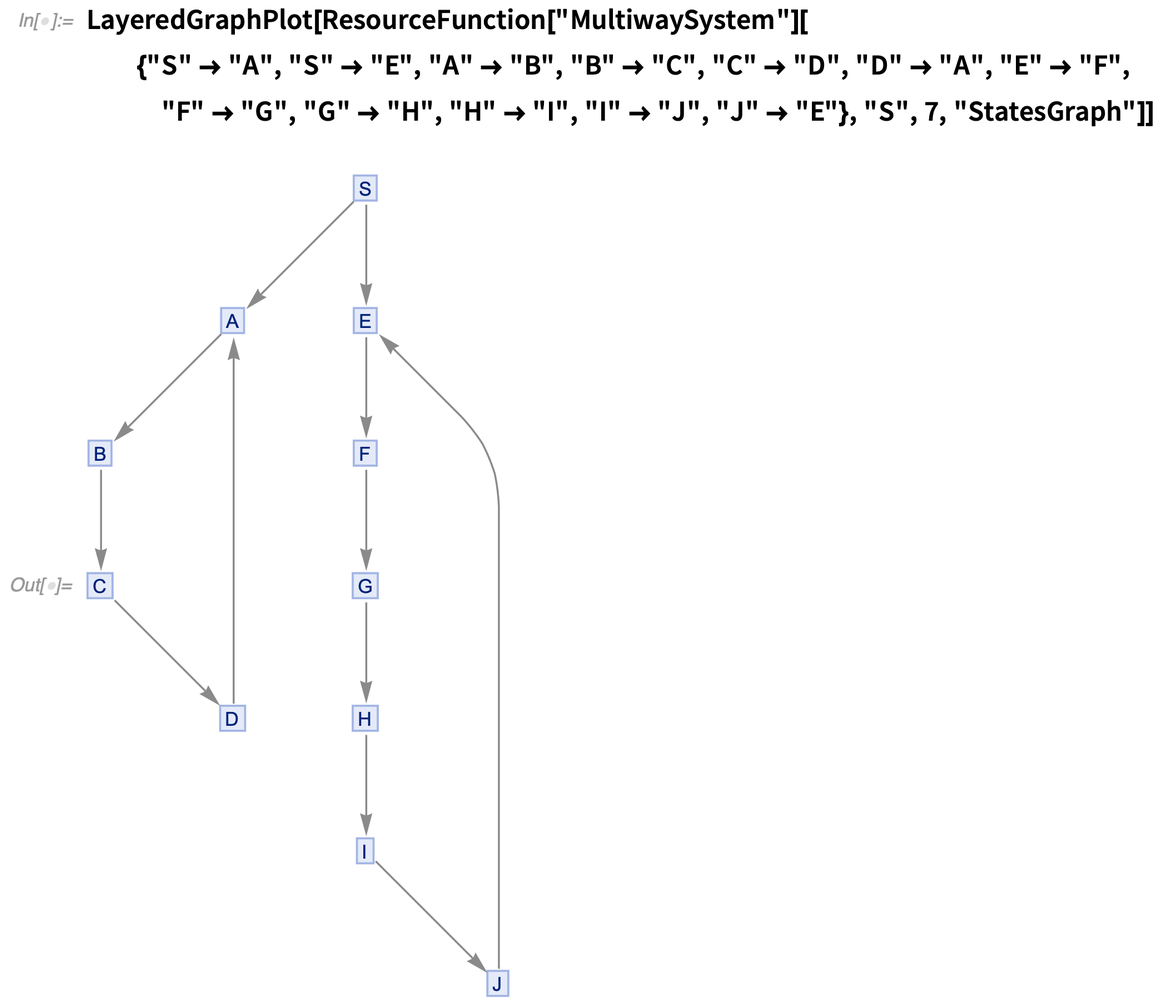✕ ```LayeredGraphPlot[ ResourceFunction["MultiwaySystem"][{"S" -> "A", "S" -> "E", "A" -> "B", "B" -> "C", "C" -> "D", "D" -> "A", "E" -> "F", "F" -> "G", "G" -> "H", "H" -> "I", "I" -> "J", "J" -> "E"}, "S", 7, "StatesGraph"]]```

Consider first the simplest nontrivial case of a multiway system containing a pair of period-2 oscillators (the states graph, illustrating the actual cycle structure, is shown on the left, while the unmerged evolution graph is shown on the right):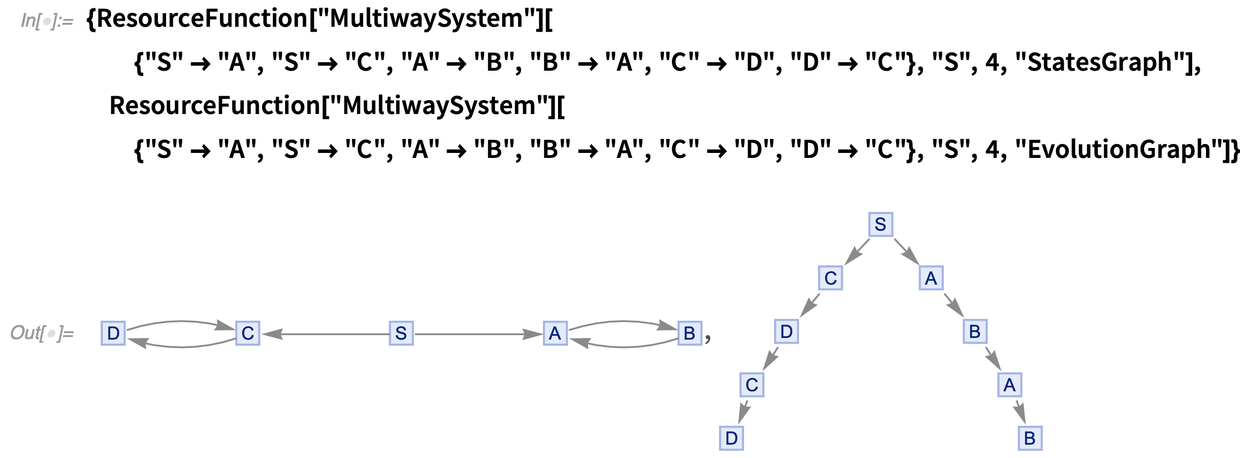✕ ```{ResourceFunction["MultiwaySystem"][{"S" -> "A", "S" -> "C", "A" -> "B", "B" -> "A", "C" -> "D", "D" -> "C"}, "S", 4, "StatesGraph"], ResourceFunction["MultiwaySystem"][{"S" -> "A", "S" -> "C", "A" -> "B", "B" -> "A", "C" -> "D", "D" -> "C"}, "S", 4, "EvolutionGraph"]}```

However, since each cycle ultimately corresponds to a single eigenstate of the associated quantum system, we can collapse each cycle down to occupy only a single state vertex in the multiway system (essentially by replacing a state with spatial periodicity 1 and temporal periodicity 2 by an equivalent state with spatial periodicity 2 and temporal periodicity 1), as follows: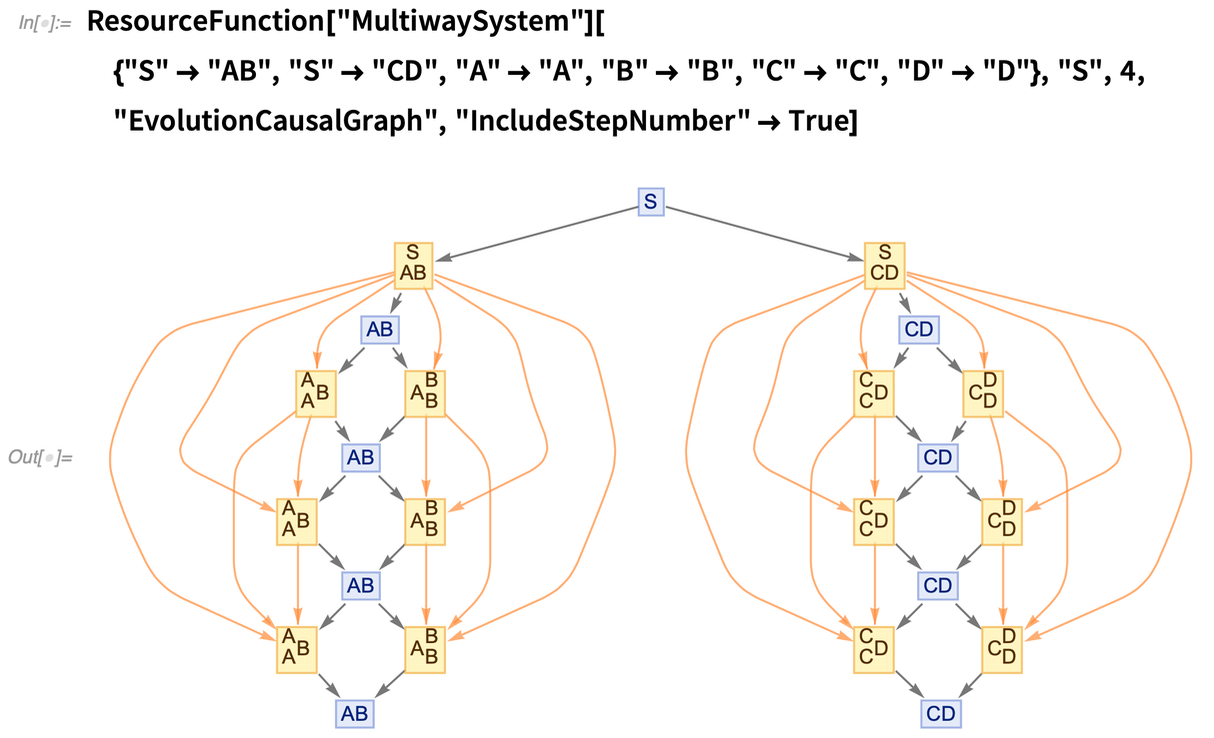✕ ```ResourceFunction["MultiwaySystem"][{"S" -> "AB", "S" -> "CD", "A" -> "A", "B" -> "B", "C" -> "C", "D" -> "D"}, "S", 4, "EvolutionCausalGraph", "IncludeStepNumber" -> True]```

Since the periods are equal between the two branches, the two oscillators are exactly in phase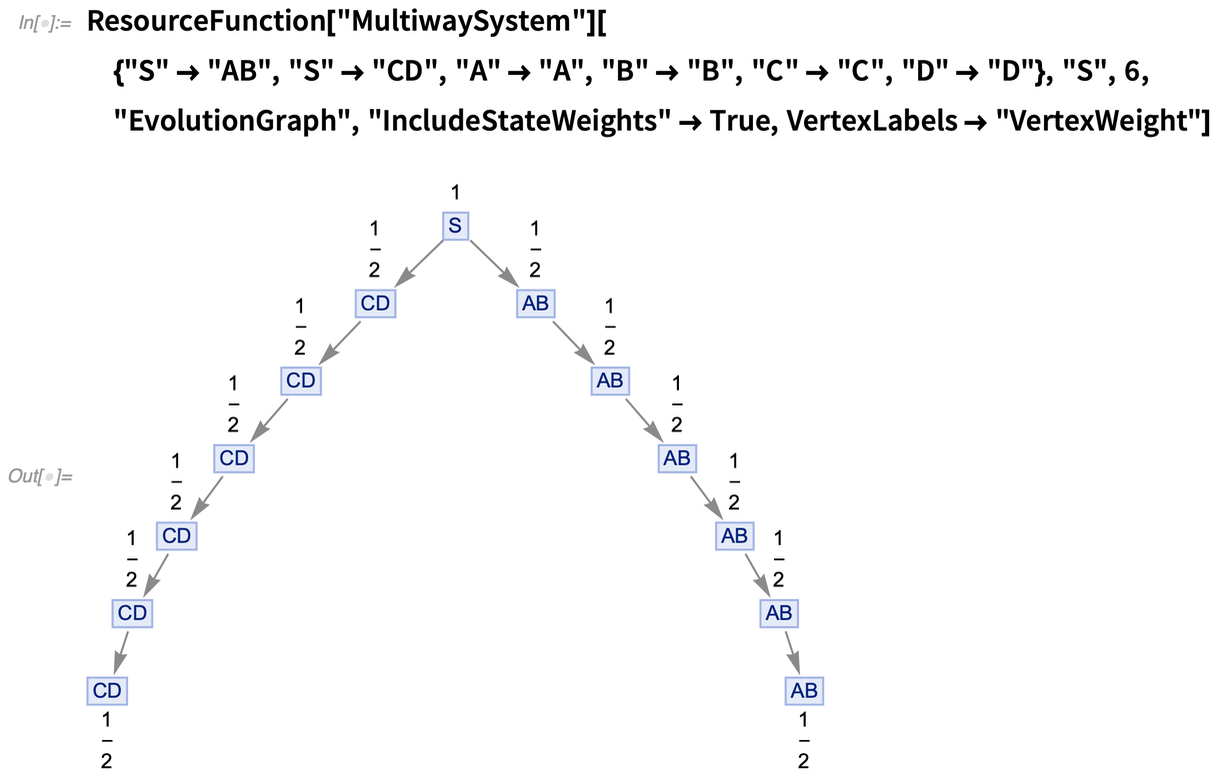✕ ```ResourceFunction["MultiwaySystem"][{"S" -> "AB", "S" -> "CD", "A" -> "A", "B" -> "B", "C" -> "C", "D" -> "D"}, "S", 6, "EvolutionGraph", "IncludeStateWeights" -> True, VertexLabels -> "VertexWeight"]```

and clearly, since the oscillators are non-interacting, the system is non-causal invariant: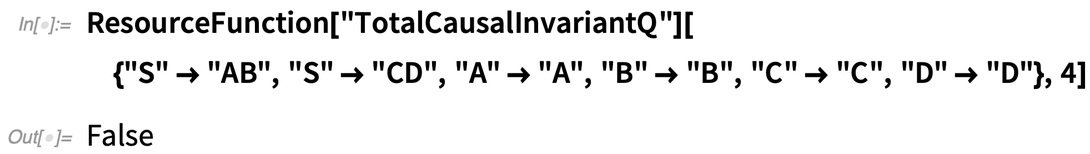✕ ```ResourceFunction["TotalCausalInvariantQ"][{"S" -> "AB", "S" -> "CD", "A" -> "A", "B" -> "B", "C" -> "C", "D" -> "D"}, 4]```

It is intuitively clear that a minimal completion (acting on single characters) would send “A”  “C” and “C”  “A”, as well as “B”  “D” and “D”  “B”. To see programmatically why this must be so, consider the set of canonical branch pairs for this particular system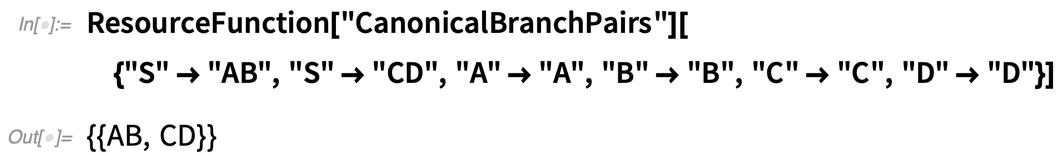✕ ```ResourceFunction["CanonicalBranchPairs"][{"S" -> "AB", "S" -> "CD", "A" -> "A", "B" -> "B", "C" -> "C", "D" -> "D"}]```

and note that the greatest common divisor of their lengths is 2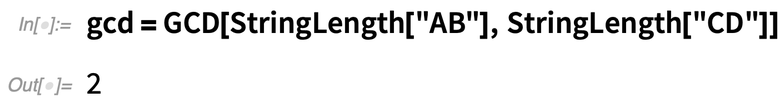✕ `gcd = GCD[StringLength["AB"], StringLength["CD"]]`

which means that we can simply construct the following direct mapping between the characters of the two strings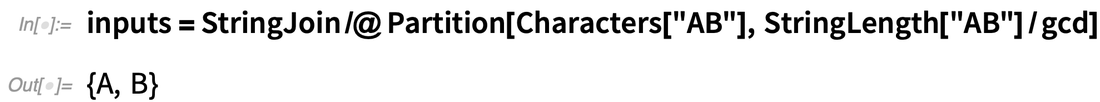✕ ```inputs = StringJoin /@ Partition[Characters["AB"], StringLength["AB"]/gcd]```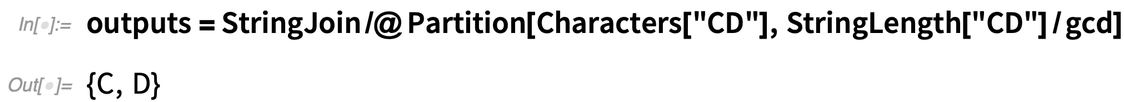✕ ```outputs = StringJoin /@ Partition[Characters["CD"], StringLength["CD"]/gcd]```

to yield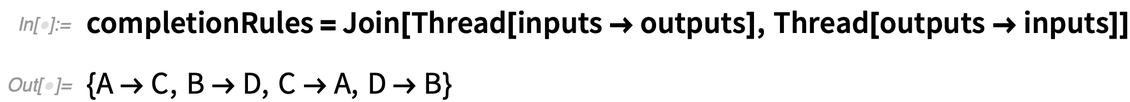✕ ```completionRules = Join[Thread[inputs -> outputs], Thread[outputs -> inputs]]```

exactly as expected. By introducing these new completion rules, we have effectively forced the two period-2 oscillators to interact, thus obtaining the following causal invariant multiway system: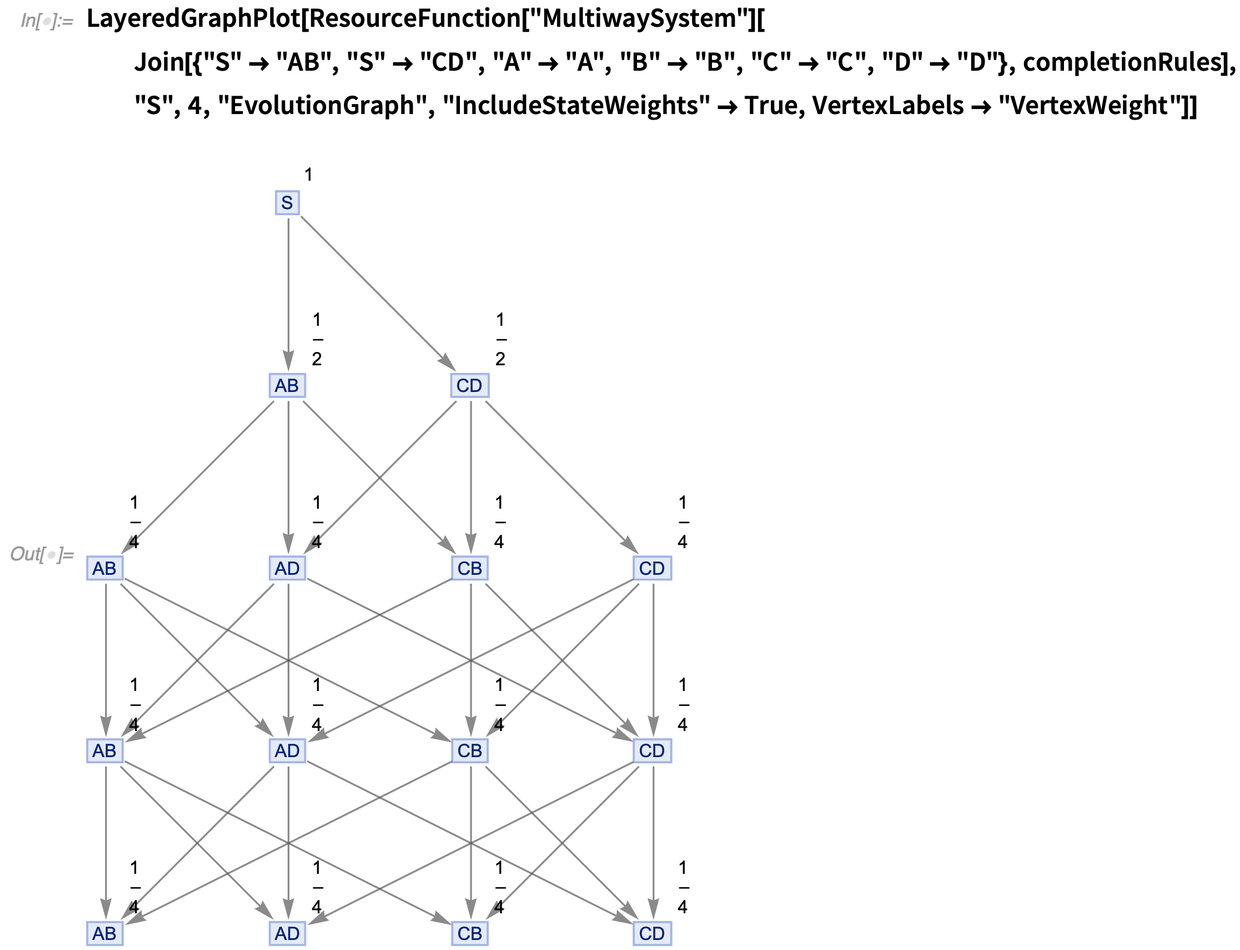✕ ```LayeredGraphPlot[ ResourceFunction["MultiwaySystem"][ Join[{"S" -> "AB", "S" -> "CD", "A" -> "A", "B" -> "B", "C" -> "C", "D" -> "D"}, completionRules], "S", 4, "EvolutionGraph", "IncludeStateWeights" -> True, VertexLabels -> "VertexWeight"]]```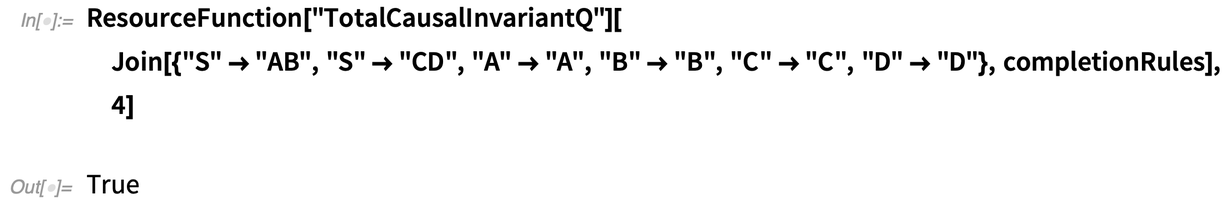✕ ```ResourceFunction["TotalCausalInvariantQ"][ Join[{"S" -> "AB", "S" -> "CD", "A" -> "A", "B" -> "B", "C" -> "C", "D" -> "D"}, completionRules], 4]```

The constructive interference of the two branches is witnessed from the fact that the state weights here remain perfectly stable post-completion: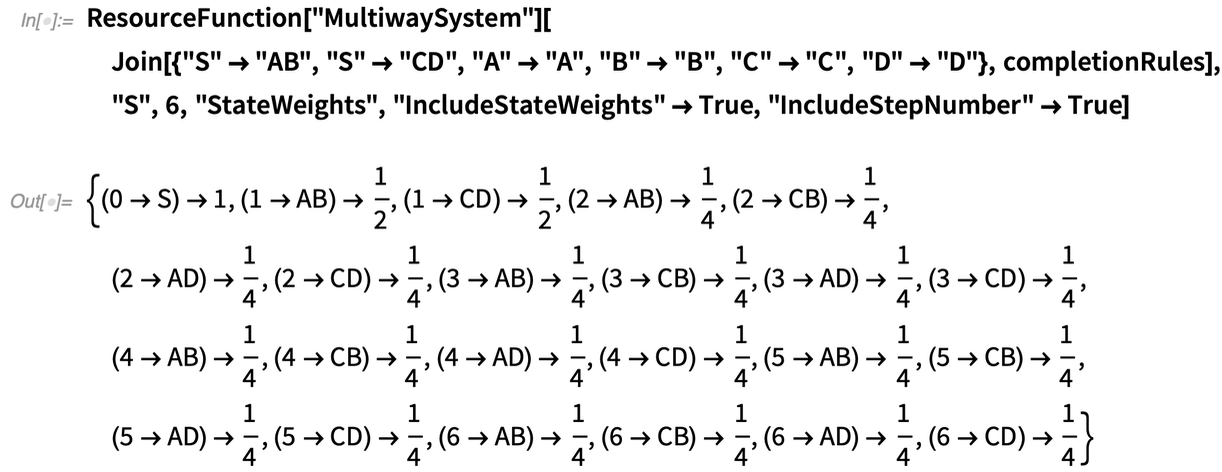✕ ```ResourceFunction["MultiwaySystem"][ Join[{"S" -> "AB", "S" -> "CD", "A" -> "A", "B" -> "B", "C" -> "C", "D" -> "D"}, completionRules], "S", 6, "StateWeights", "IncludeStateWeights" -> True, "IncludeStepNumber" -> True]```

Consider now a multiway system consisting of a period-2 oscillator and a period-3 oscillator, i.e.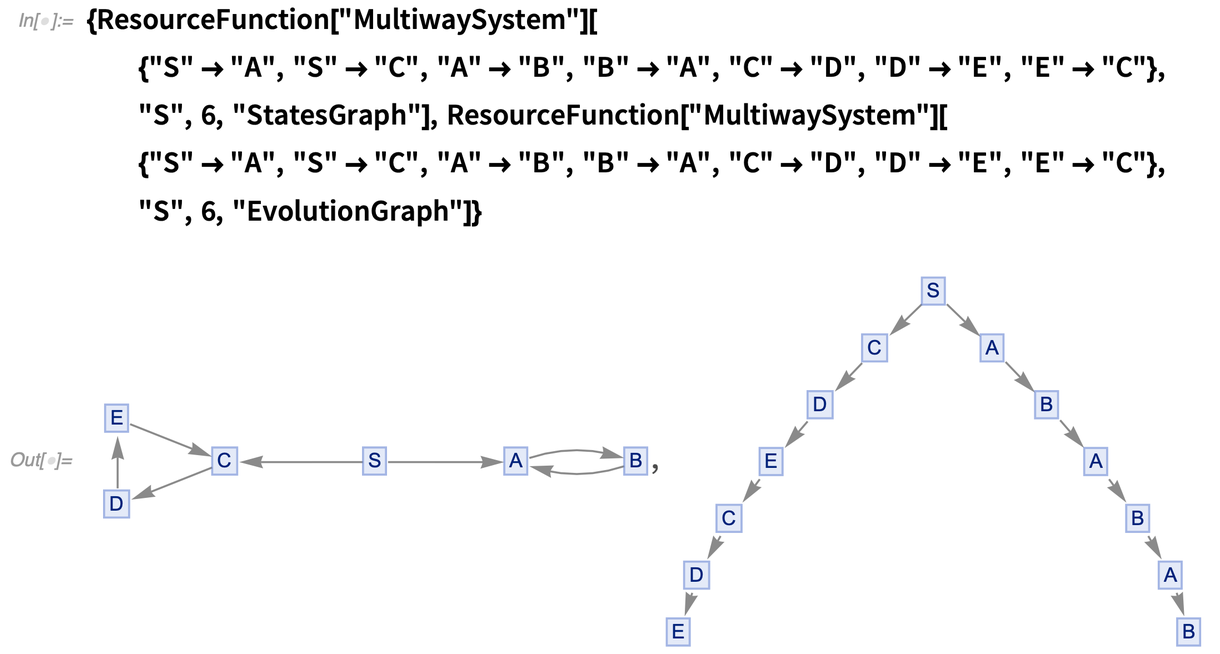✕ ```{ResourceFunction["MultiwaySystem"][{"S" -> "A", "S" -> "C", "A" -> "B", "B" -> "A", "C" -> "D", "D" -> "E", "E" -> "C"}, "S", 6, "StatesGraph"], ResourceFunction["MultiwaySystem"][{"S" -> "A", "S" -> "C", "A" -> "B", "B" -> "A", "C" -> "D", "D" -> "E", "E" -> "C"}, "S", 6, "EvolutionGraph"]}```

and collapse the eigenstates down to occupy single multiway states in the usual way: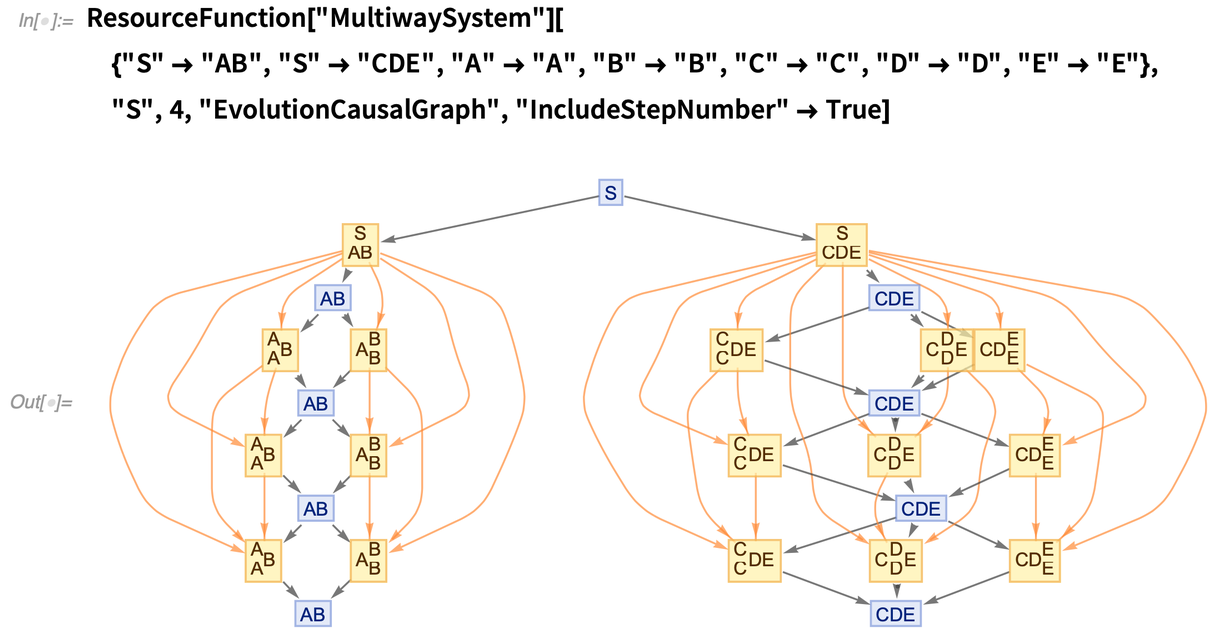✕ ```ResourceFunction["MultiwaySystem"][{"S" -> "AB", "S" -> "CDE", "A" -> "A", "B" -> "B", "C" -> "C", "D" -> "D", "E" -> "E"}, "S", 4, "EvolutionCausalGraph", "IncludeStepNumber" -> True]```

Since the periods of the two oscillators differ by a factor of 1/2, we can see that the two multiway branches are therefore exactly π radians out of phase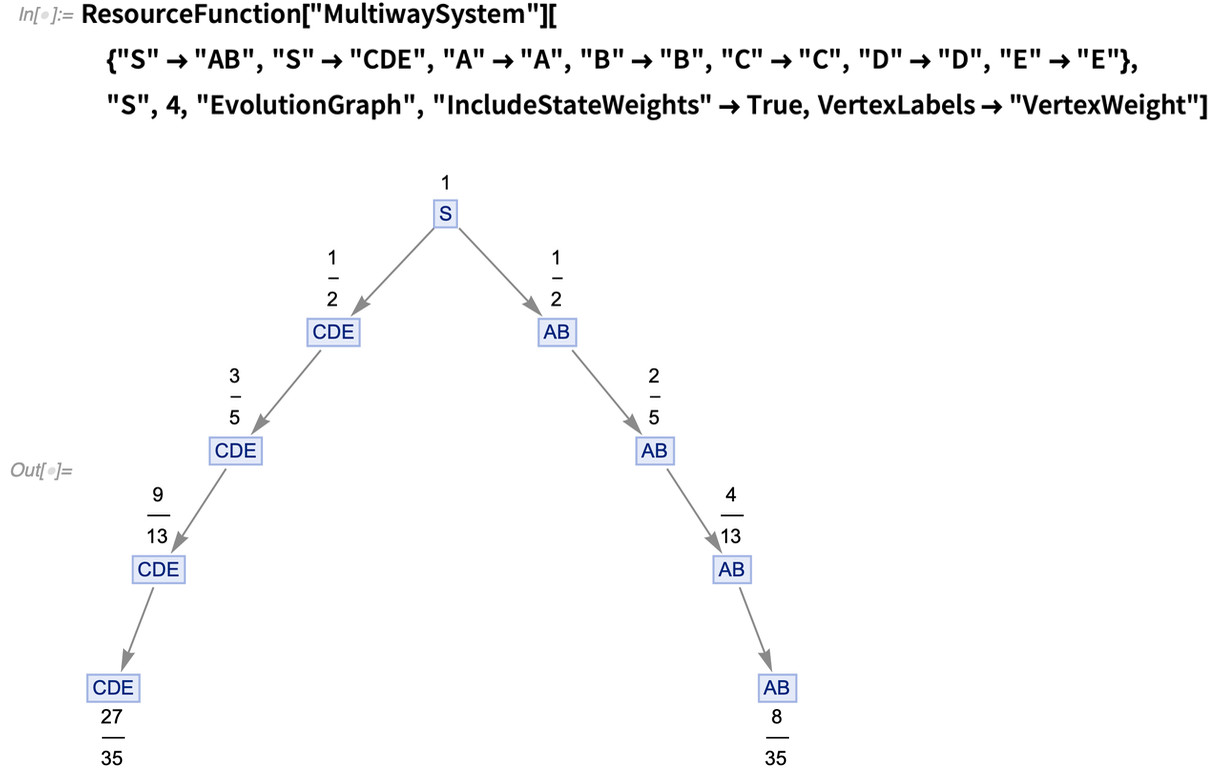✕ ```ResourceFunction["MultiwaySystem"][{"S" -> "AB", "S" -> "CDE", "A" -> "A", "B" -> "B", "C" -> "C", "D" -> "D", "E" -> "E"}, "S", 4, "EvolutionGraph", "IncludeStateWeights" -> True, VertexLabels -> "VertexWeight"]```

and, since it has not been measured, the system is not yet causal invariant: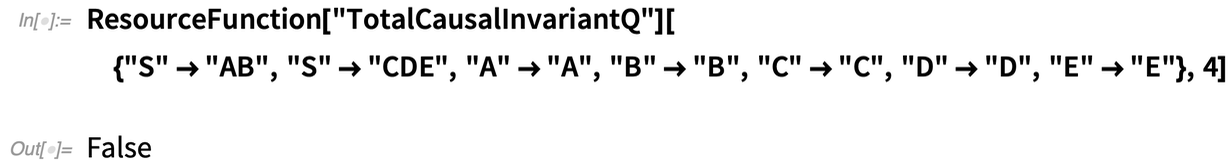✕ ```ResourceFunction["TotalCausalInvariantQ"][{"S" -> "AB", "S" -> "CDE", "A" -> "A", "B" -> "B", "C" -> "C", "D" -> "D", "E" -> "E"}, 4]```

Due to the mismatch between the periods of the two branches, it is no longer immediately clear what the minimal (single-character) completion procedure should be. Following the same basic algorithm we employed previously, begin by considering the set of canonical branch pairs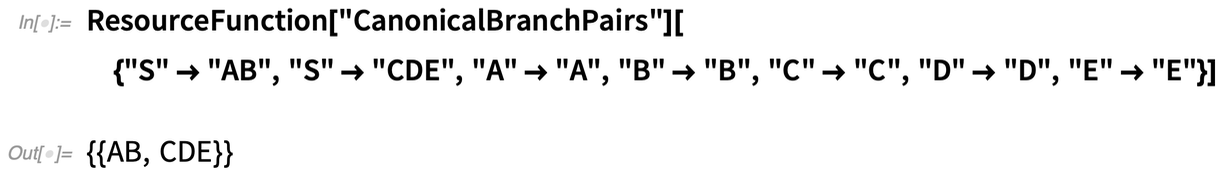✕ ```ResourceFunction["CanonicalBranchPairs"][{"S" -> "AB", "S" -> "CDE", "A" -> "A", "B" -> "B", "C" -> "C", "D" -> "D", "E" -> "E"}]```

and observe this time around that the greatest common divisor of their lengths is 1 (i.e. the lengths are coprime)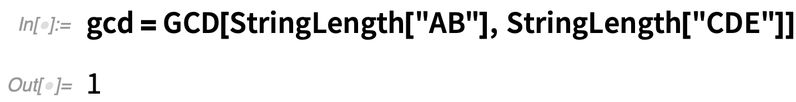✕ `gcd = GCD[StringLength["AB"], StringLength["CDE"]]`

so if we attempt to construct the same naive mapping between the characters of the two strings as before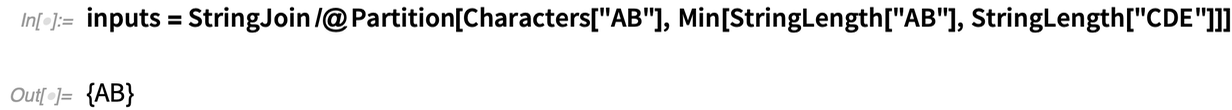✕ ```inputs = StringJoin /@ Partition[Characters["AB"], Min[StringLength["AB"], StringLength["CDE"]]]```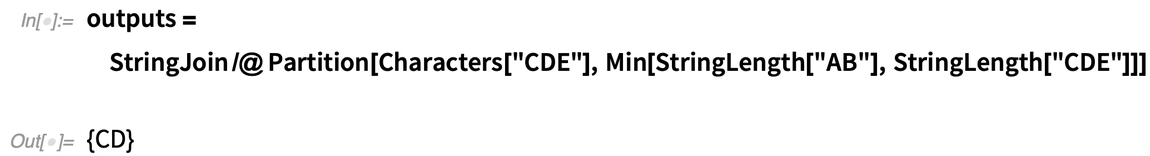✕ ```outputs = StringJoin /@ Partition[Characters["CDE"], Min[StringLength["AB"], StringLength["CDE"]]]```

then we obtain only a partial set of completion rules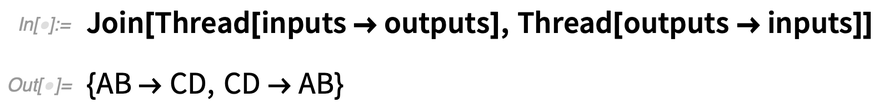✕ `Join[Thread[inputs -> outputs], Thread[outputs -> inputs]]`

since the substring “E” is still unaccounted for. Therefore, we must also incorporate an additional pair of rules allowing one to map from the end of the “AB” string (i.e. the empty string) to the end of the “CDE” string (i.e. the “E” substring), which we can do using elementary modular arithmetic. More precisely, the final 3 (i.e. the string length of “CDE”) modulo 2 (i.e. the string length of “AB”) characters of the “CDE” string must be mapped onto the empty string that terminates “AB”, and vice versa, i.e.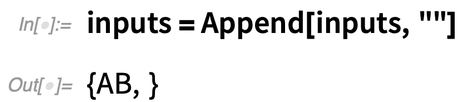✕ `inputs = Append[inputs, ""]`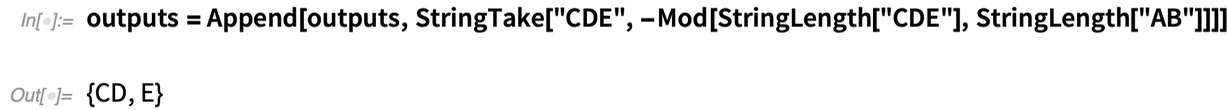✕ ```outputs = Append[outputs, StringTake["CDE", -Mod[StringLength["CDE"], StringLength["AB"]]]]```

to obtain the overall set of completion rules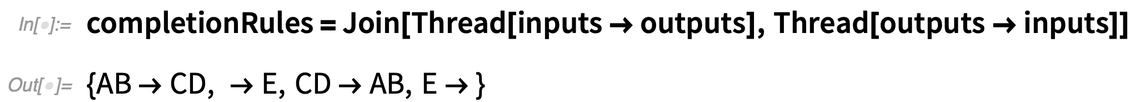✕ ```completionRules = Join[Thread[inputs -> outputs], Thread[outputs -> inputs]]```

thus yielding a causal invariant multiway system in the expected manner, by effectively forcing the two out-of-phase oscillators to interact: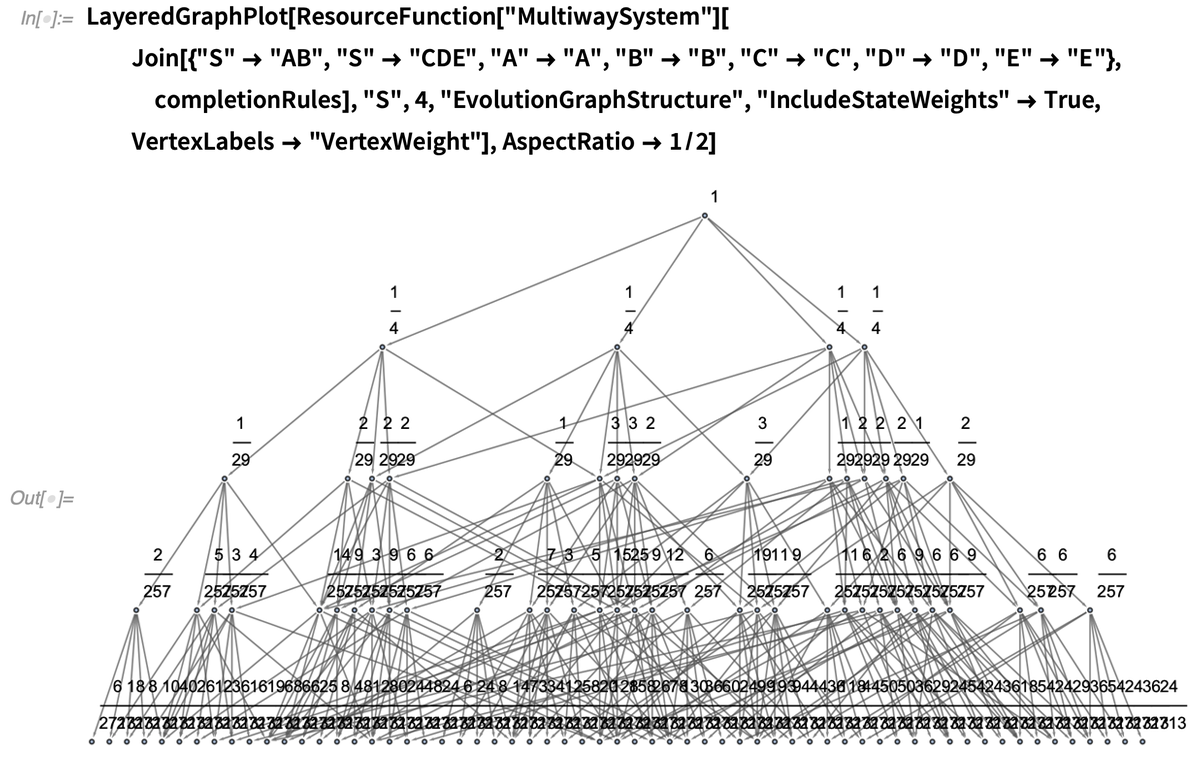✕ ```LayeredGraphPlot[ ResourceFunction["MultiwaySystem"][ Join[{"S" -> "AB", "S" -> "CDE", "A" -> "A", "B" -> "B", "C" -> "C", "D" -> "D", "E" -> "E"}, completionRules], "S", 4, "EvolutionGraphStructure", "IncludeStateWeights" -> True, VertexLabels -> "VertexWeight"], AspectRatio -> 1/2]```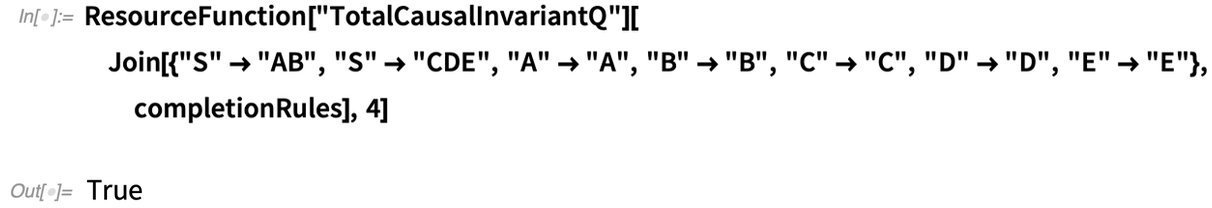✕ ```ResourceFunction["TotalCausalInvariantQ"][ Join[{"S" -> "AB", "S" -> "CDE", "A" -> "A", "B" -> "B", "C" -> "C", "D" -> "D", "E" -> "E"}, completionRules], 4]```

However, note that the state weights (post-completion), unlike in the earlier constructive case, are now unstable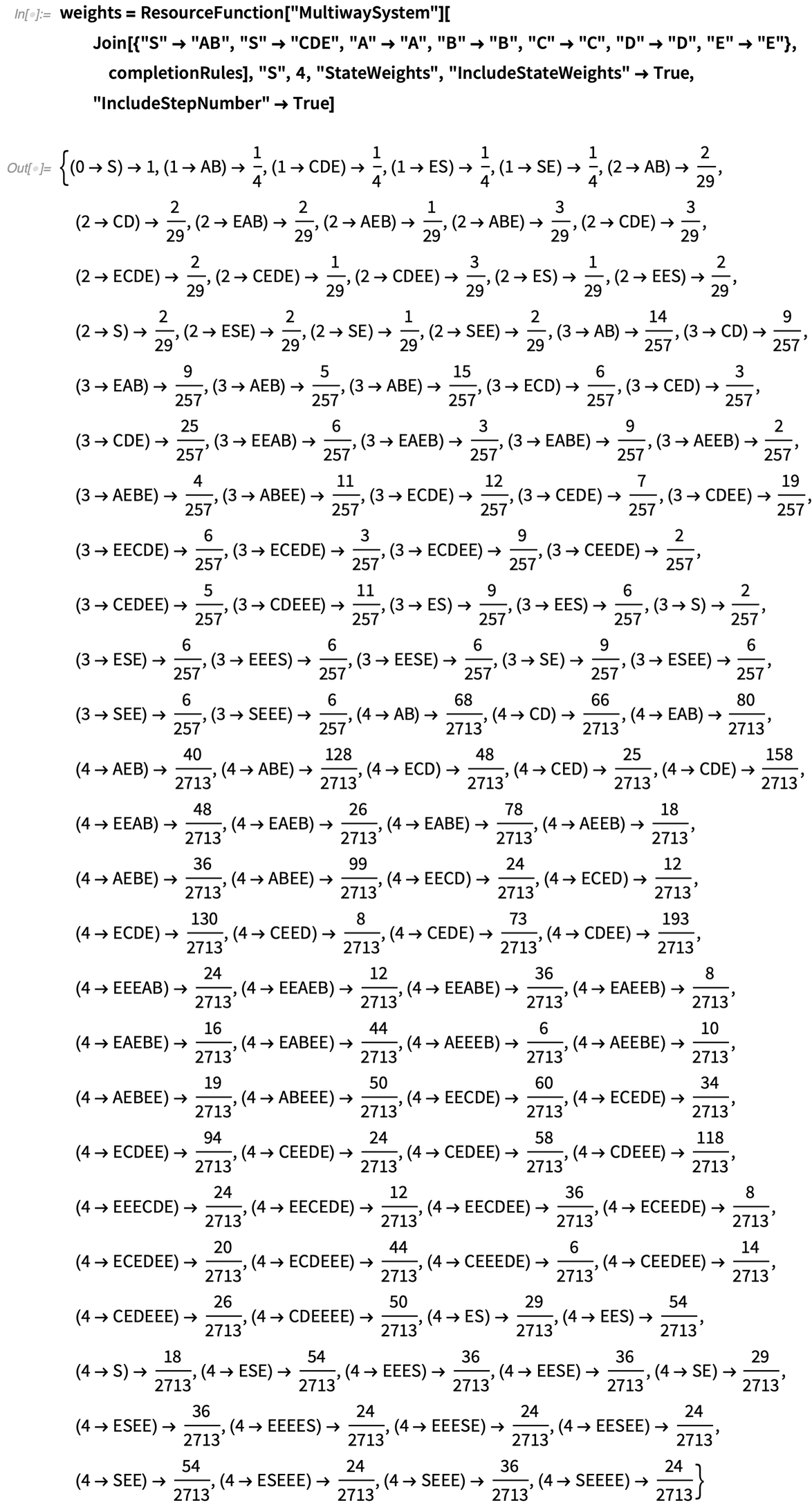✕ ```weights = ResourceFunction["MultiwaySystem"][ Join[{"S" -> "AB", "S" -> "CDE", "A" -> "A", "B" -> "B", "C" -> "C", "D" -> "D", "E" -> "E"}, completionRules], "S", 4, "StateWeights", "IncludeStateWeights" -> True, "IncludeStepNumber" -> True]```

and that, in particular, the weights associated with states “AB” and “CDE” appear to be converging to zero, corresponding to the phenomenon of perfect destructive interference: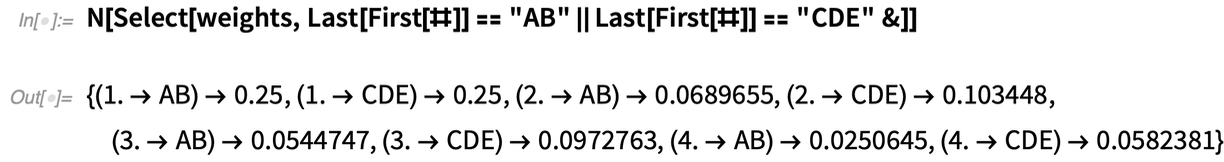✕ ```N[Select[weights, Last[First[#]] == "AB" || Last[First[#]] == "CDE" &]]```

We can validate this convergence empirically by simply running the multiway system for a couple more evolution steps: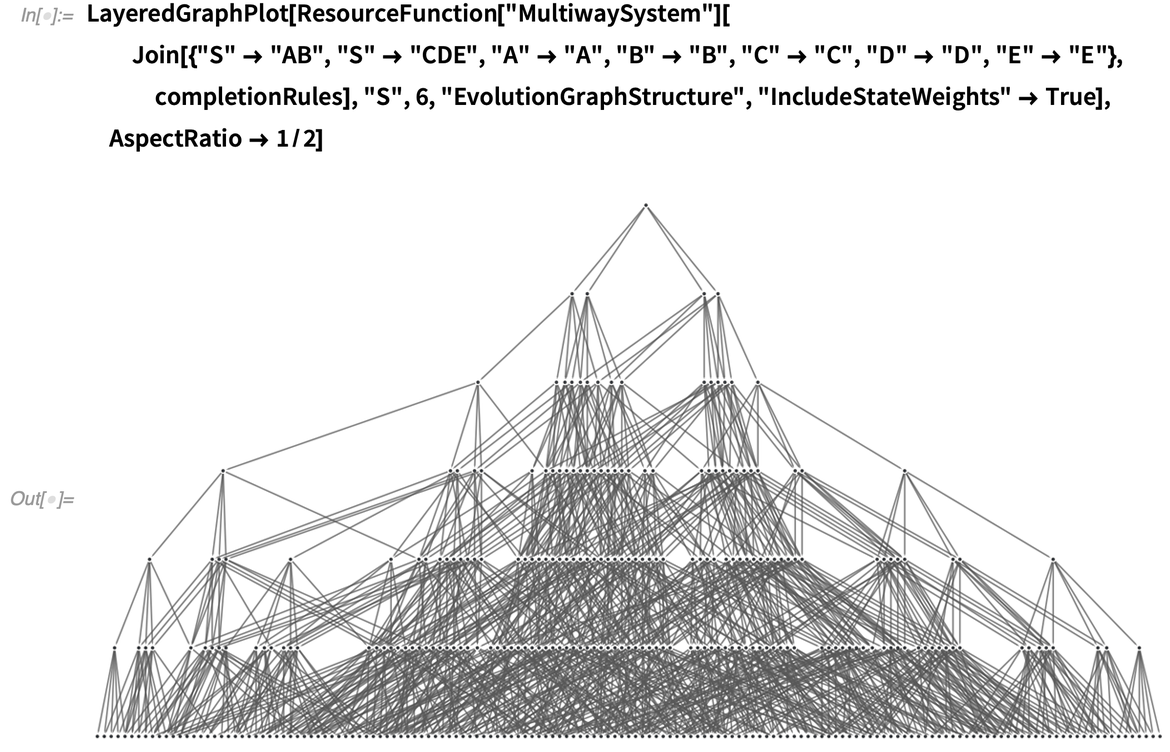✕ ```LayeredGraphPlot[ ResourceFunction["MultiwaySystem"][ Join[{"S" -> "AB", "S" -> "CDE", "A" -> "A", "B" -> "B", "C" -> "C", "D" -> "D", "E" -> "E"}, completionRules], "S", 6, "EvolutionGraphStructure", "IncludeStateWeights" -> True], AspectRatio -> 1/2]```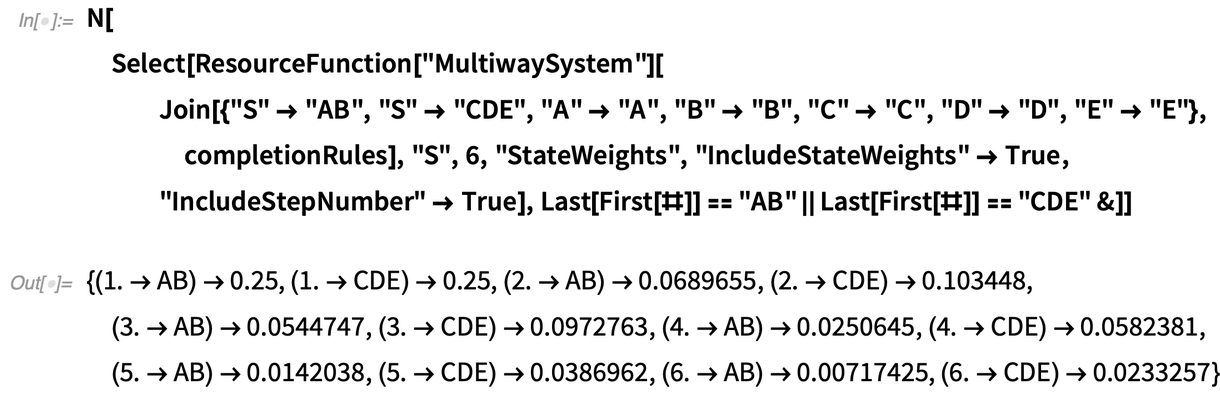✕ ```N[Select[ResourceFunction["MultiwaySystem"][ Join[{"S" -> "AB", "S" -> "CDE", "A" -> "A", "B" -> "B", "C" -> "C", "D" -> "D", "E" -> "E"}, completionRules], "S", 6, "StateWeights", "IncludeStateWeights" -> True, "IncludeStepNumber" -> True], Last[First[#]] == "AB" || Last[First[#]] == "CDE" &]]```

As a final illustrative step, let us consider a multiway system containing a period-2 oscillator and a period-4 oscillator, as shown here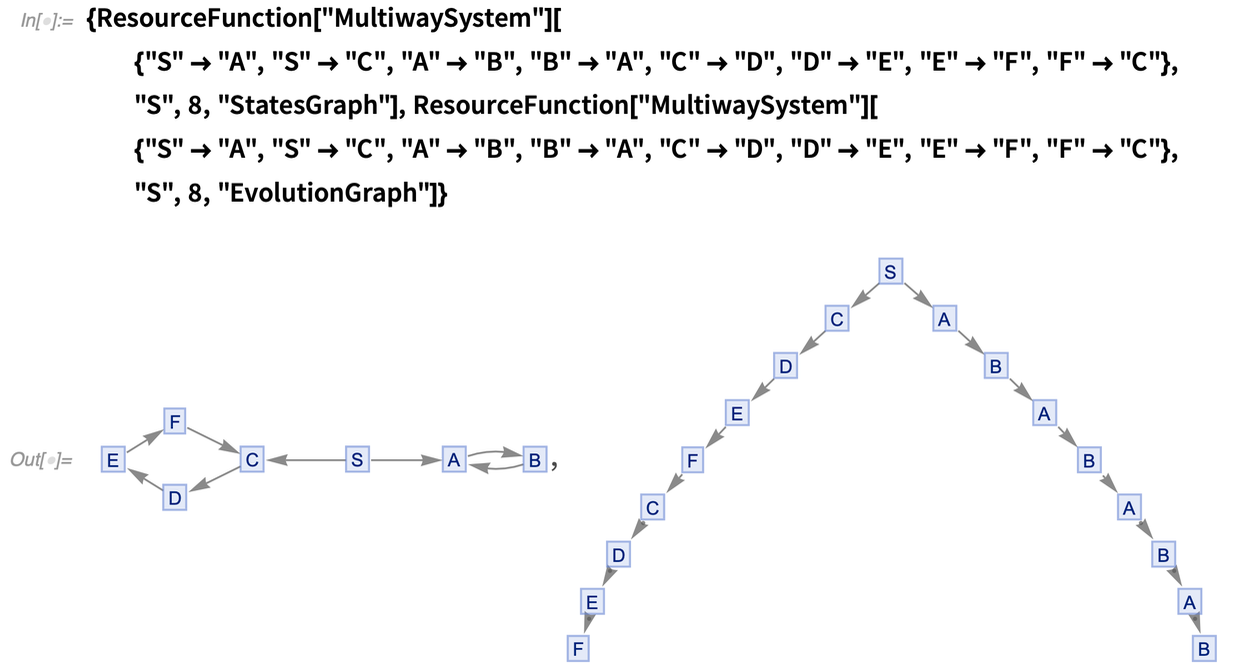✕ ```{ResourceFunction["MultiwaySystem"][{"S" -> "A", "S" -> "C", "A" -> "B", "B" -> "A", "C" -> "D", "D" -> "E", "E" -> "F", "F" -> "C"}, "S", 8, "StatesGraph"], ResourceFunction["MultiwaySystem"][{"S" -> "A", "S" -> "C", "A" -> "B", "B" -> "A", "C" -> "D", "D" -> "E", "E" -> "F", "F" -> "C"}, "S", 8, "EvolutionGraph"]}```

which, with the eigenstates collapsed down to occupy single multiway states, now looks like this: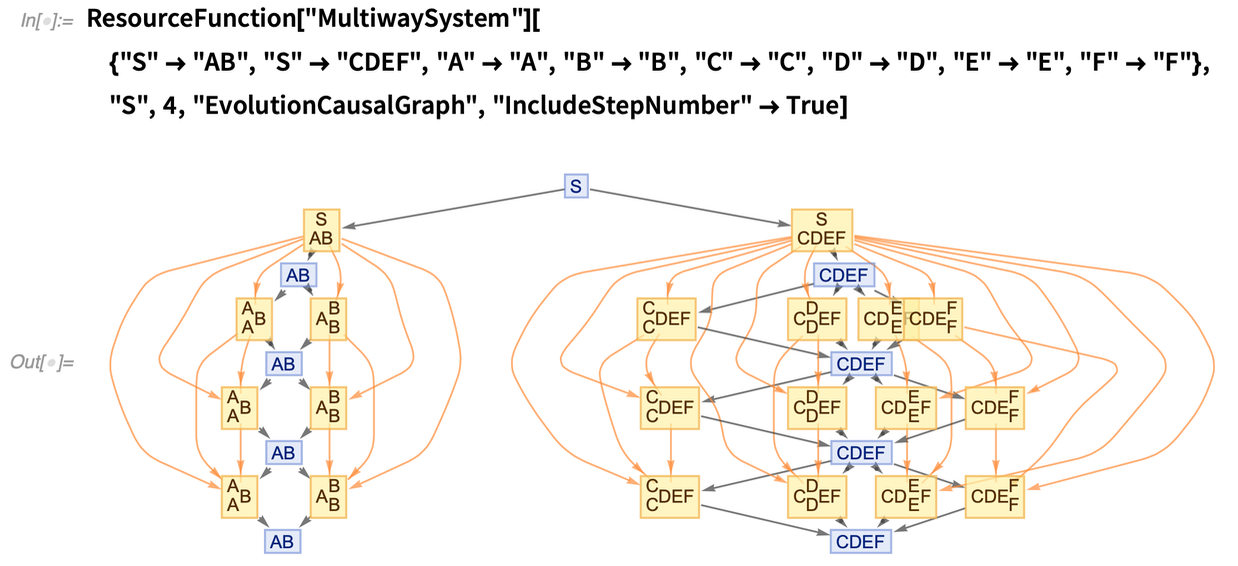✕ ```ResourceFunction["MultiwaySystem"][{"S" -> "AB", "S" -> "CDEF", "A" -> "A", "B" -> "B", "C" -> "C", "D" -> "D", "E" -> "E", "F" -> "F"}, "S", 4, "EvolutionCausalGraph", "IncludeStepNumber" -> True]```

The periods of the two oscillators thus differ by exactly 2*π, so the two branches are now back in phase with each other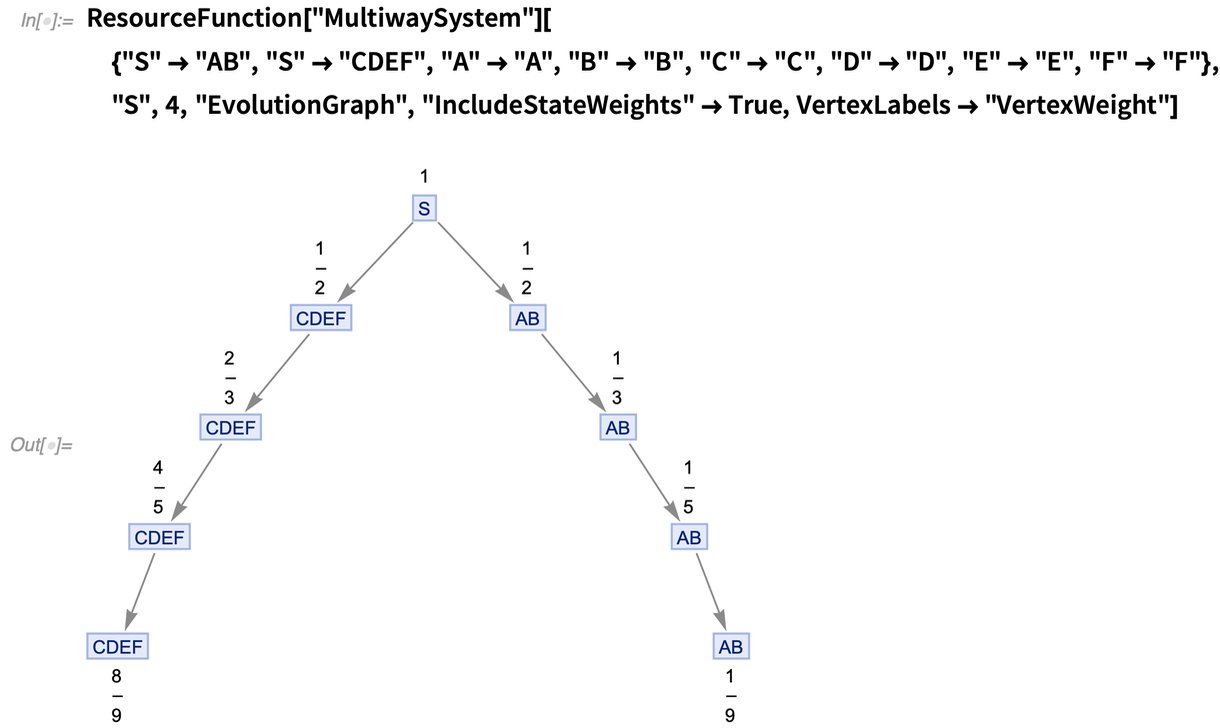✕ ```ResourceFunction["MultiwaySystem"][{"S" -> "AB", "S" -> "CDEF", "A" -> "A", "B" -> "B", "C" -> "C", "D" -> "D", "E" -> "E", "F" -> "F"}, "S", 4, "EvolutionGraph", "IncludeStateWeights" -> True, VertexLabels -> "VertexWeight"]```

and, as usual, the unmeasured system fails to be causal invariant: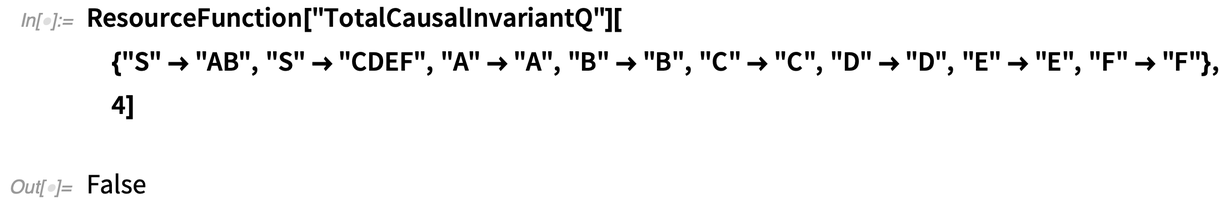✕ ```ResourceFunction["TotalCausalInvariantQ"][{"S" -> "AB", "S" -> "CDEF", "A" -> "A", "B" -> "B", "C" -> "C", "D" -> "D", "E" -> "E", "F" -> "F"}, 4]```

Thanks to the exact divisibility of the period of one oscillator by the period of the other, it is clear (by analogy with the earlier case of the two branches with equal periods) that a minimal single-character completion for this case would send “A”  “CD”, “B”  “EF”, in addition to “CD”  “A” and “EF”  “B”, which we can also confirm programmatically by simply computing the list of branch pairs: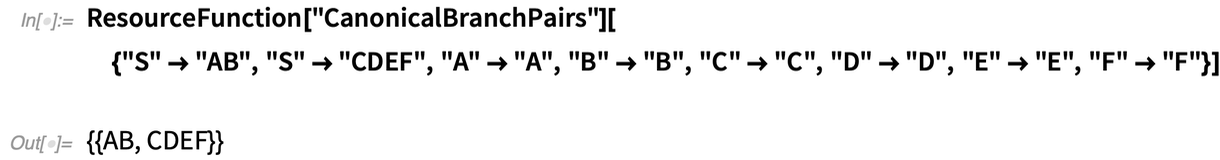✕ ```ResourceFunction["CanonicalBranchPairs"][{"S" -> "AB", "S" -> "CDEF", "A" -> "A", "B" -> "B", "C" -> "C", "D" -> "D", "E" -> "E", "F" -> "F"}]```

Since the greatest common divisor of their lengths is, again, 2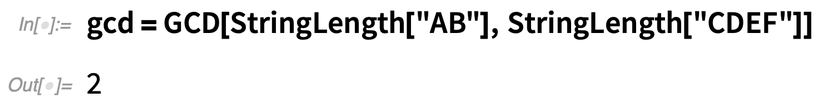✕ `gcd = GCD[StringLength["AB"], StringLength["CDEF"]]`

we are able to construct the following direct mapping between the characters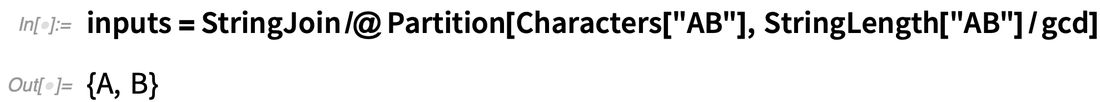✕ ```inputs = StringJoin /@ Partition[Characters["AB"], StringLength["AB"]/gcd]```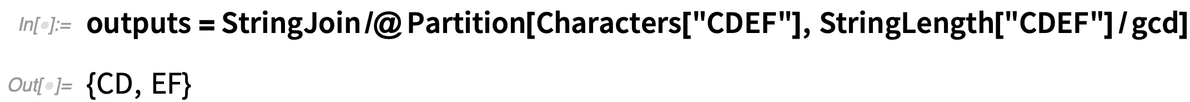✕ ```outputs = StringJoin /@ Partition[Characters["CDEF"], StringLength["CDEF"]/gcd]```

so as to obtain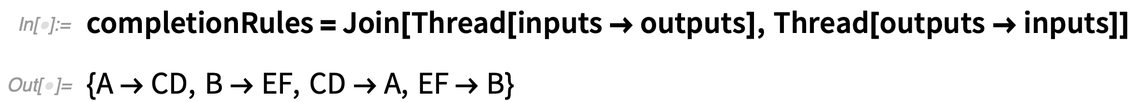✕ ```completionRules = Join[Thread[inputs -> outputs], Thread[outputs -> inputs]]```

as we had anticipated, such that the (now causal invariant) multiway system for the pair of interacting oscillators, post-completion, takes the form: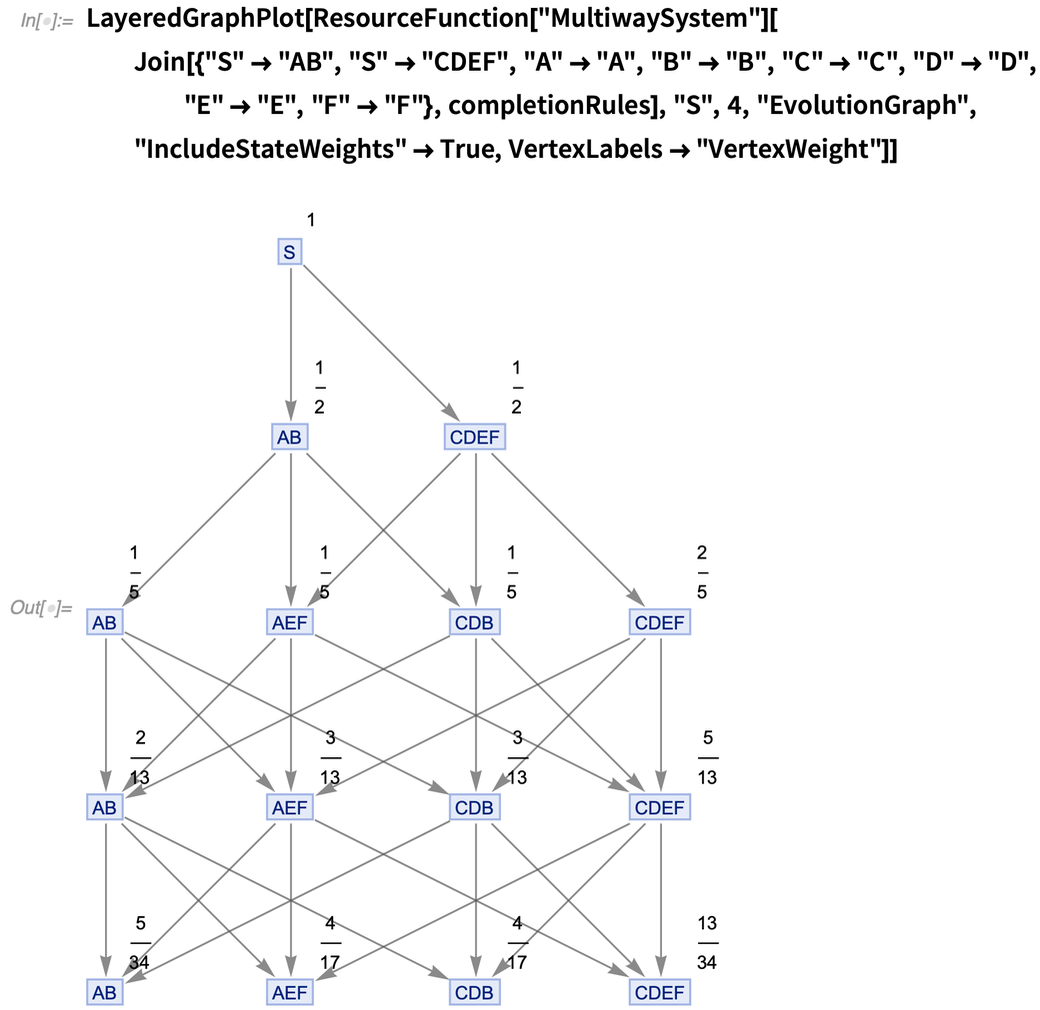✕ ```LayeredGraphPlot[ ResourceFunction["MultiwaySystem"][ Join[{"S" -> "AB", "S" -> "CDEF", "A" -> "A", "B" -> "B", "C" -> "C", "D" -> "D", "E" -> "E", "F" -> "F"}, completionRules], "S", 4, "EvolutionGraph", "IncludeStateWeights" -> True, VertexLabels -> "VertexWeight"]]```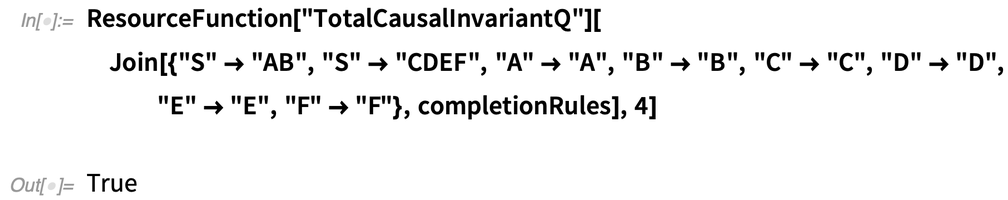✕ ```ResourceFunction["TotalCausalInvariantQ"][ Join[{"S" -> "AB", "S" -> "CDEF", "A" -> "A", "B" -> "B", "C" -> "C", "D" -> "D", "E" -> "E", "F" -> "F"}, completionRules], 4]```

The new state weights remain stable, just as in the aforementioned constructive interference case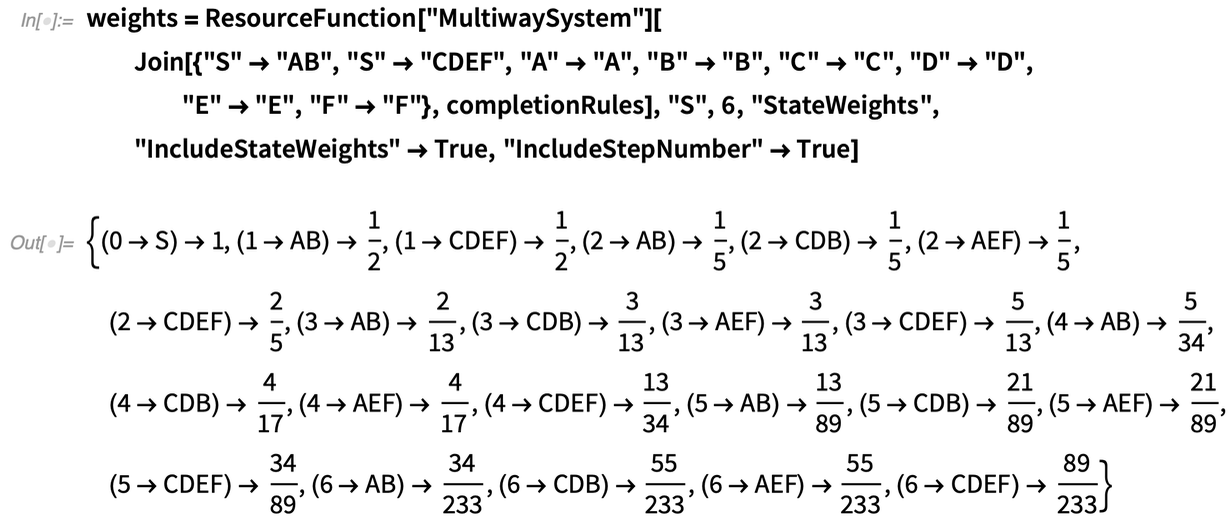✕ ```weights = ResourceFunction["MultiwaySystem"][ Join[{"S" -> "AB", "S" -> "CDEF", "A" -> "A", "B" -> "B", "C" -> "C", "D" -> "D", "E" -> "E", "F" -> "F"}, completionRules], "S", 6, "StateWeights", "IncludeStateWeights" -> True, "IncludeStepNumber" -> True]```

such that, in particular, the weights associated with states “AB” and “CDEF” now converge to finite (nonzero) values, unlike in the destructive example previously shown: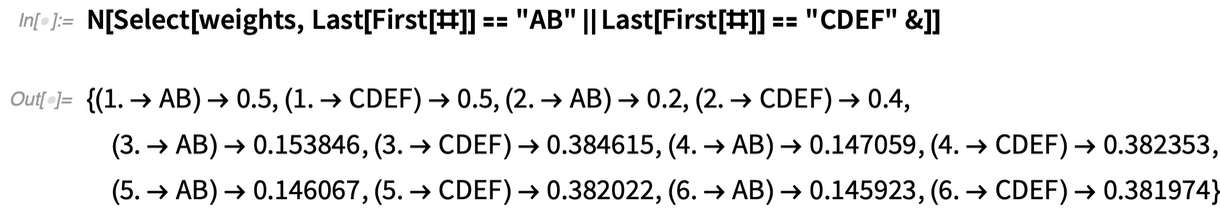✕ ```N[Select[weights, Last[First[#]] == "AB" || Last[First[#]] == "CDEF" &]]```

A more rigorous version of this argument using elementary combinatorial number theory is currently under preparation for submission as a short supplementary mathematical note to this bulletin.

Posted in: Physics